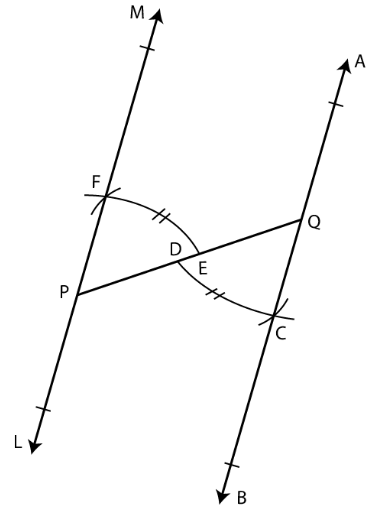# Selina Solutions Concise Maths Class 7 Chapter 14: Lines and Angles (Including Construction of Angles)

Selina Solutions Concise Maths Class 7 Chapter 14 Lines and Angles (Including Construction of Angles) are designed by highly knowledgeable faculty at BYJU’S, keeping in mind the understanding capacity of students. The PDF of solutions prepare students to solve problems without any difficulty. It also improves confidence among students to appear for the annual exam. Selina Solutions Concise Maths Class 7 Chapter 14 Lines and Angles (Including Construction of Angles), PDF links are given here, with a free download option.

Chapter 14 deals with basic terms, types of lines, types of angles and the construction of angles. In the solutions, students are provided with the steps to be followed in the construction of various structures.

## Selina Solutions Concise Maths Class 7 Chapter 14: Lines and Angles (Including Construction of Angles) Download PDF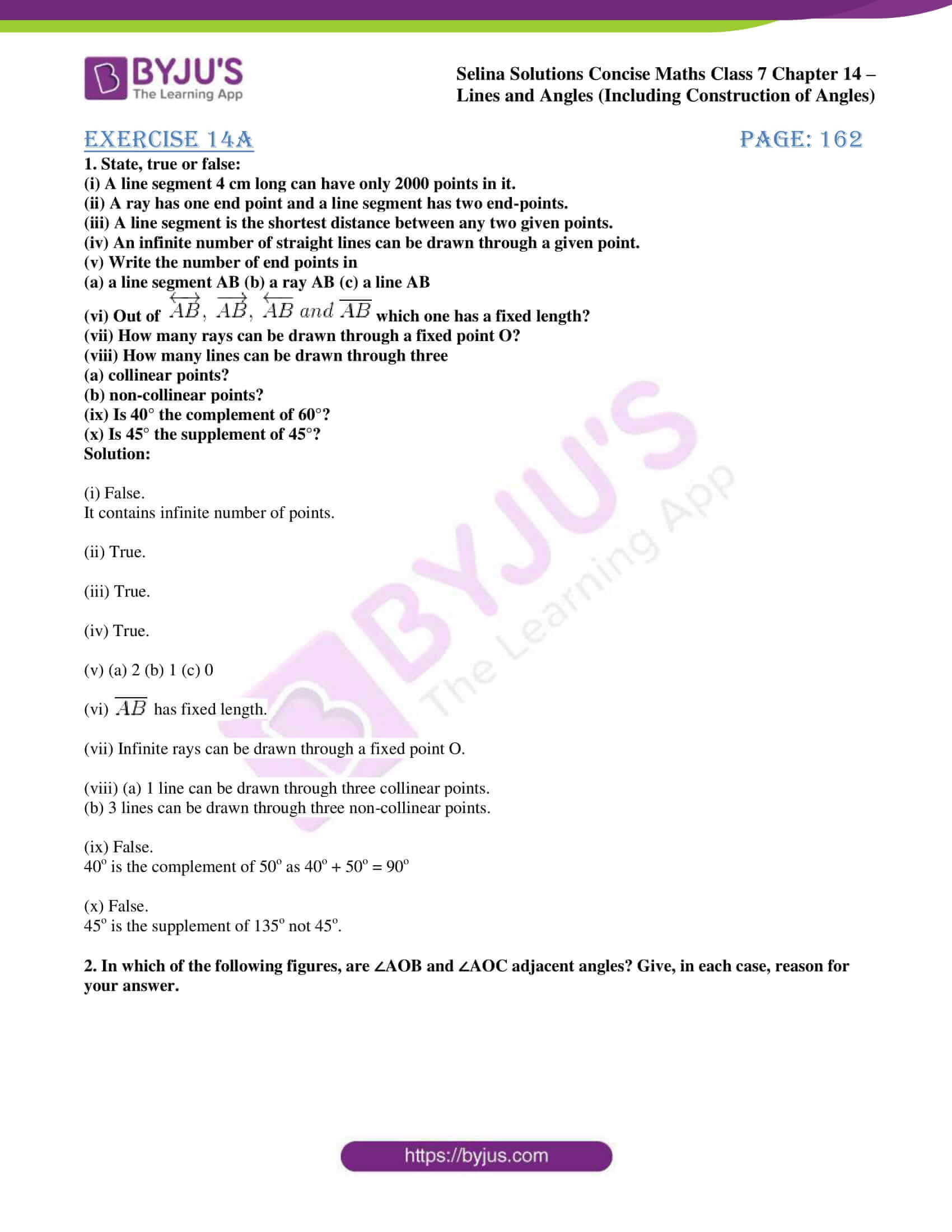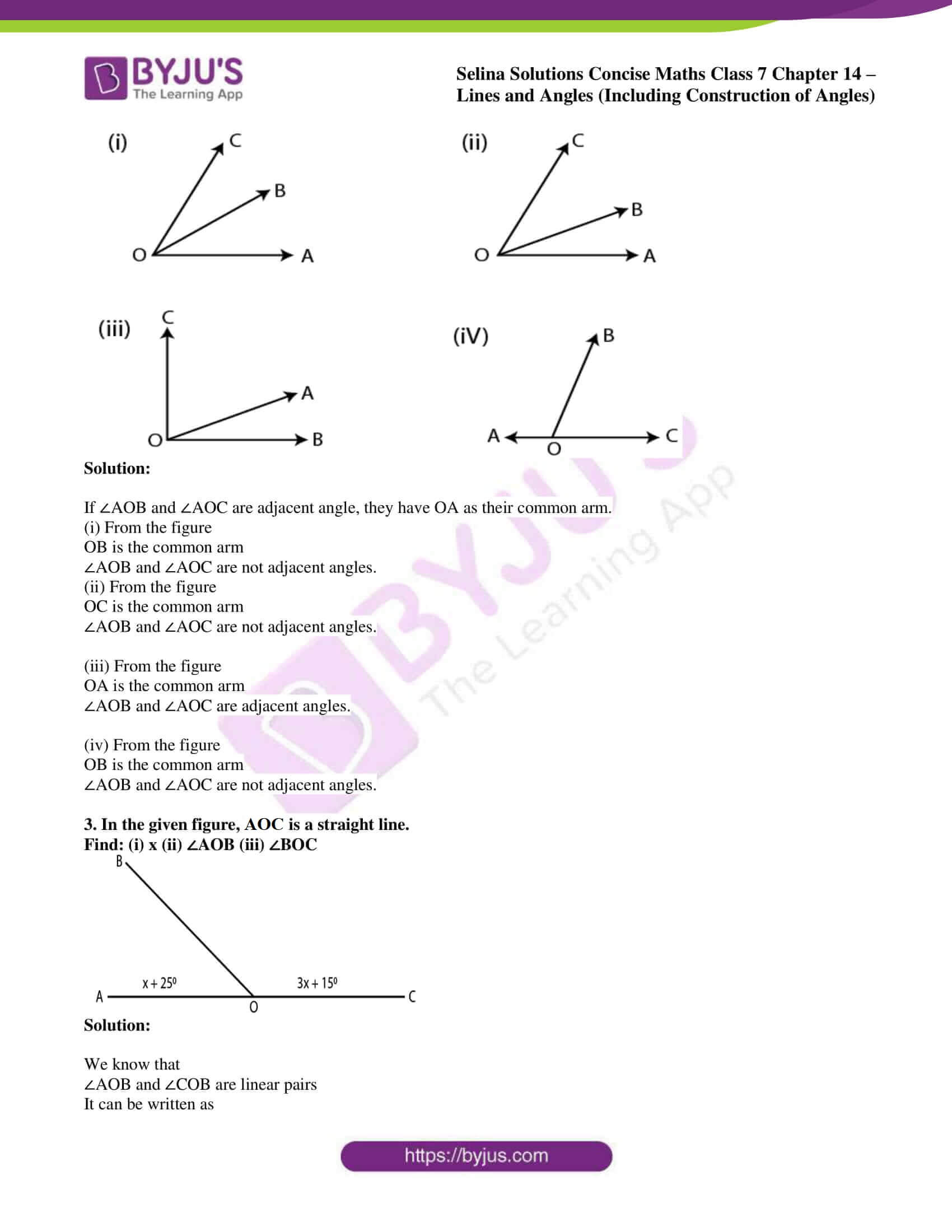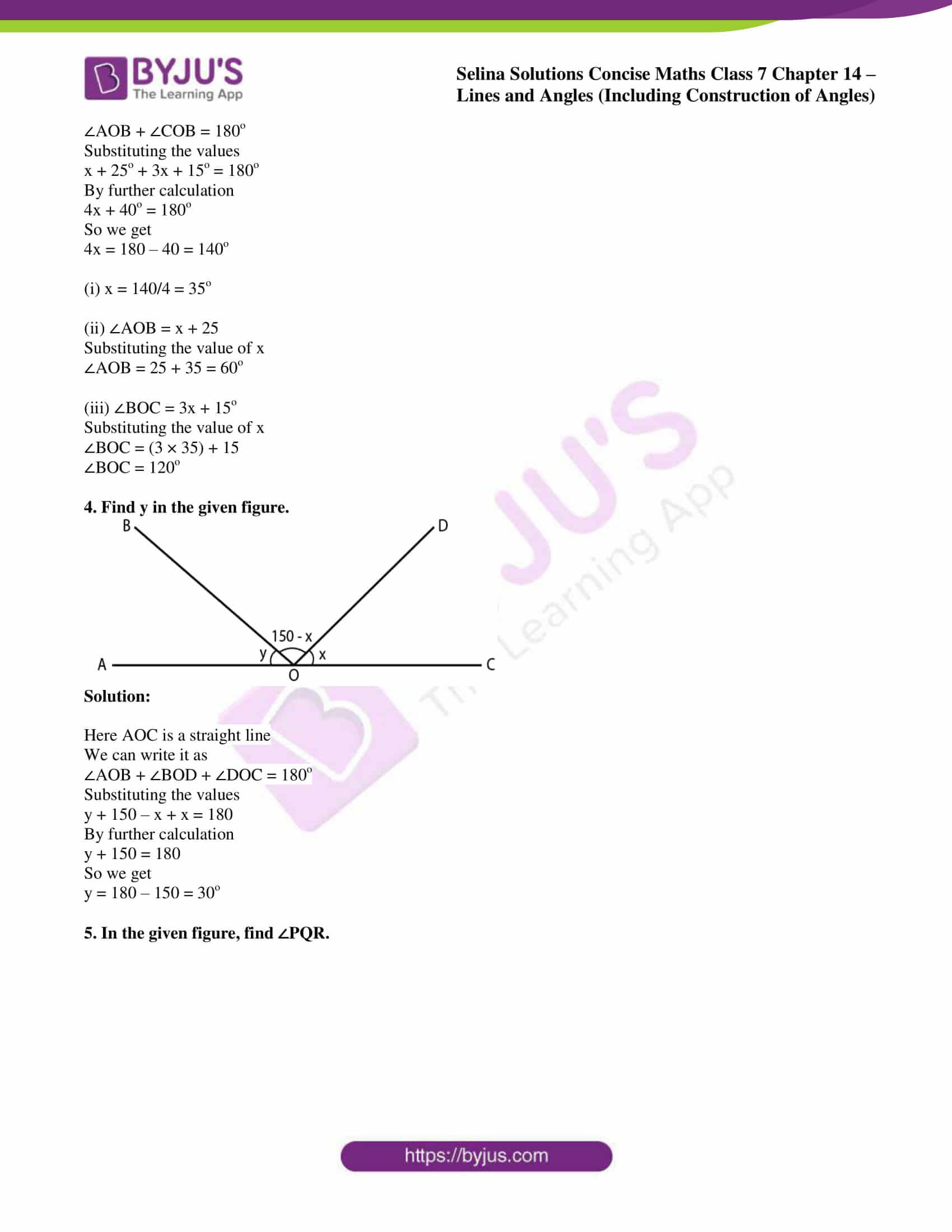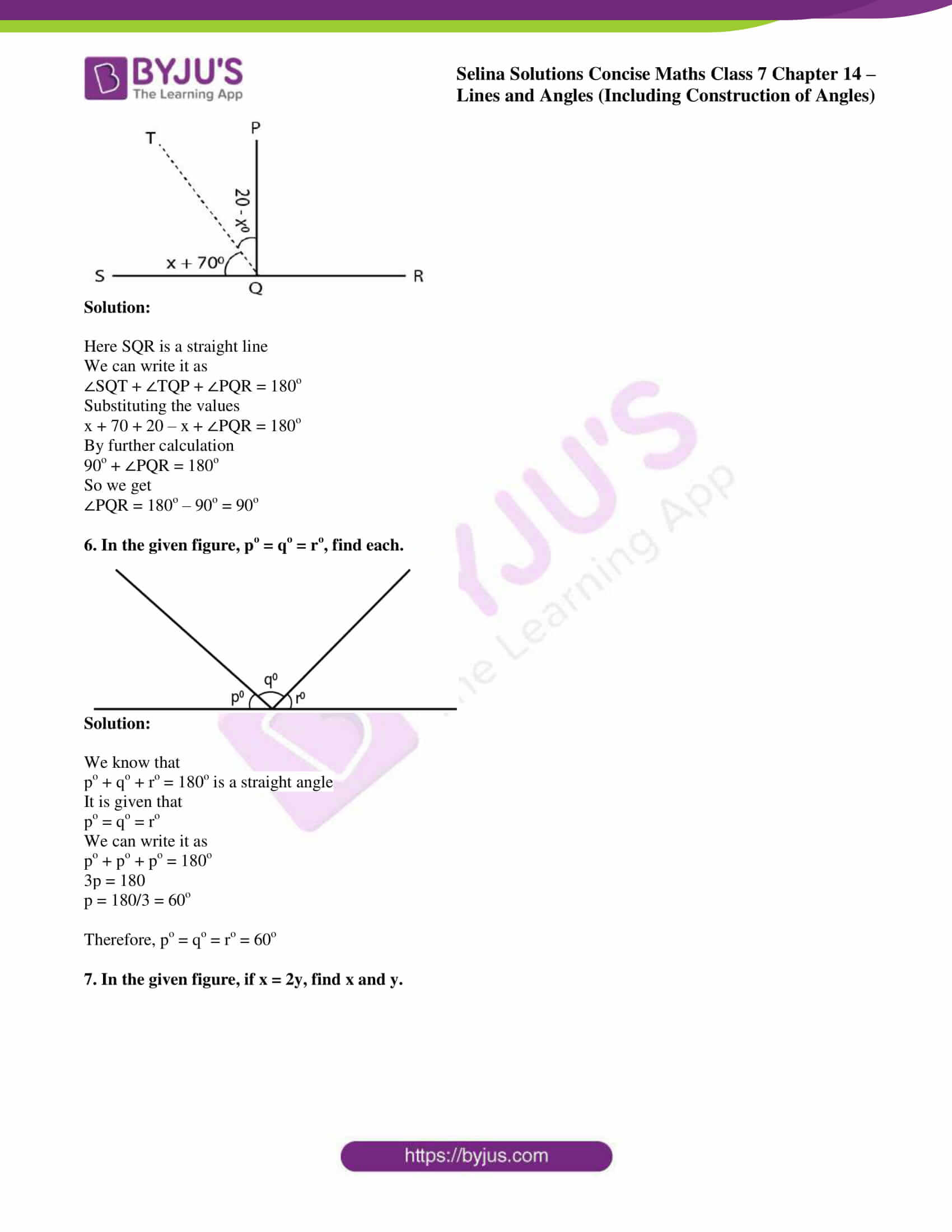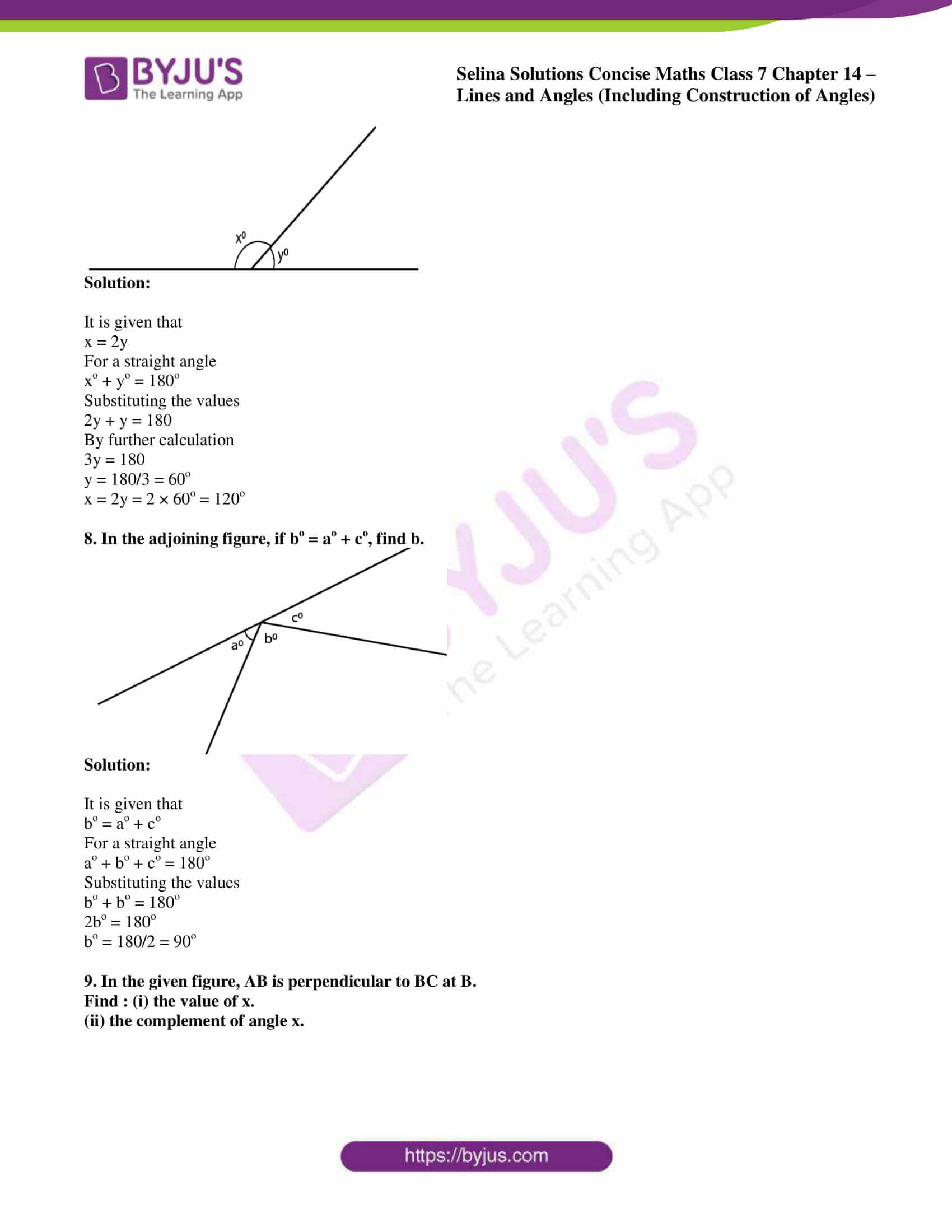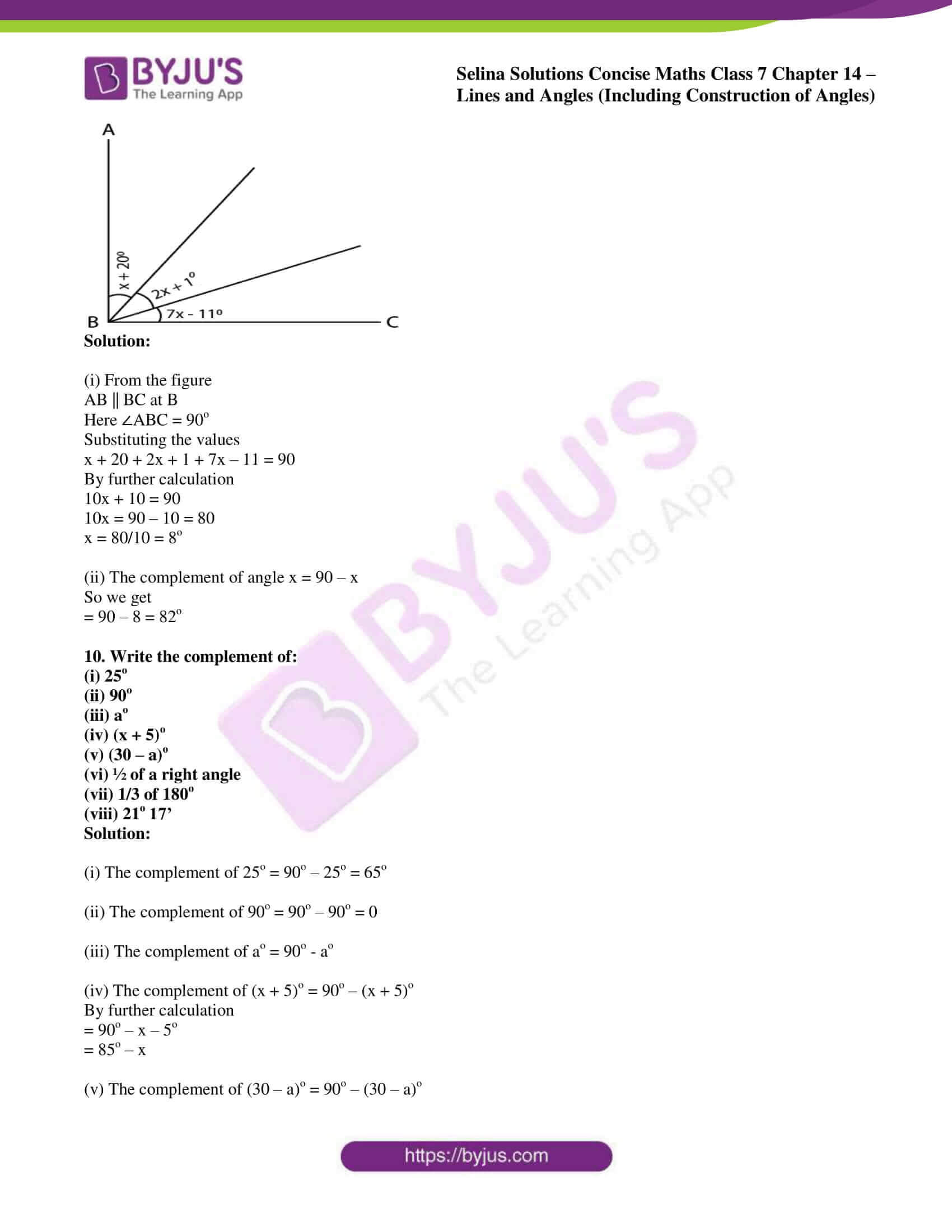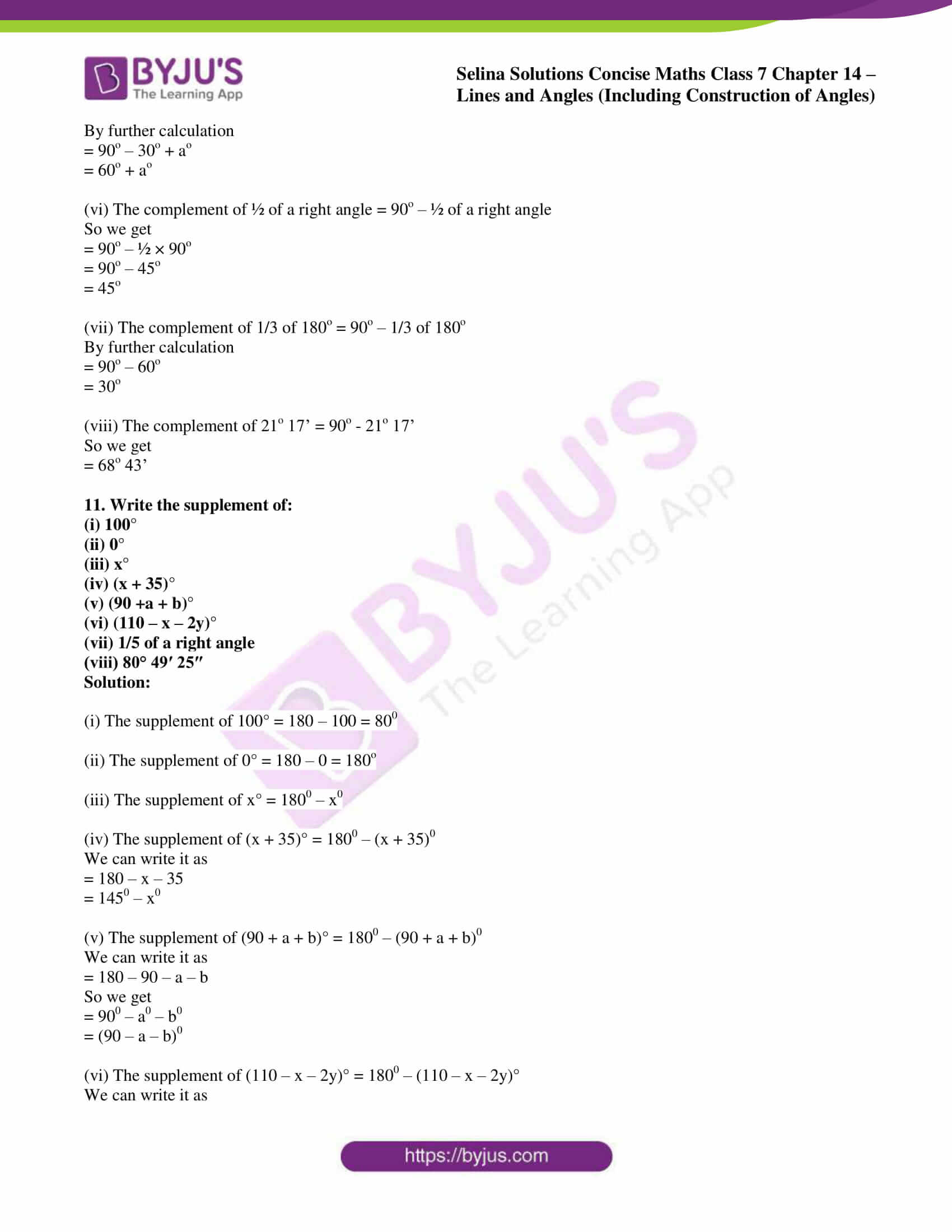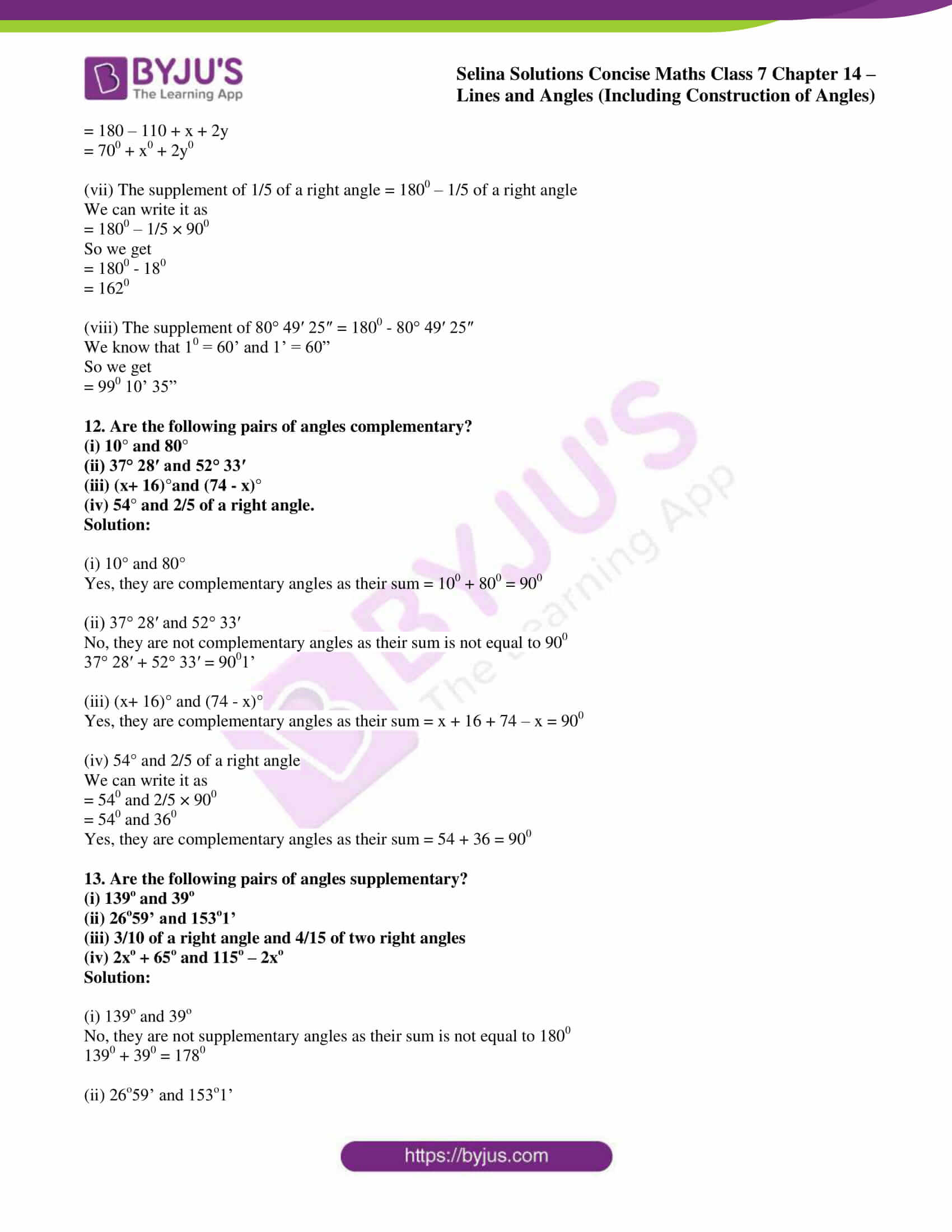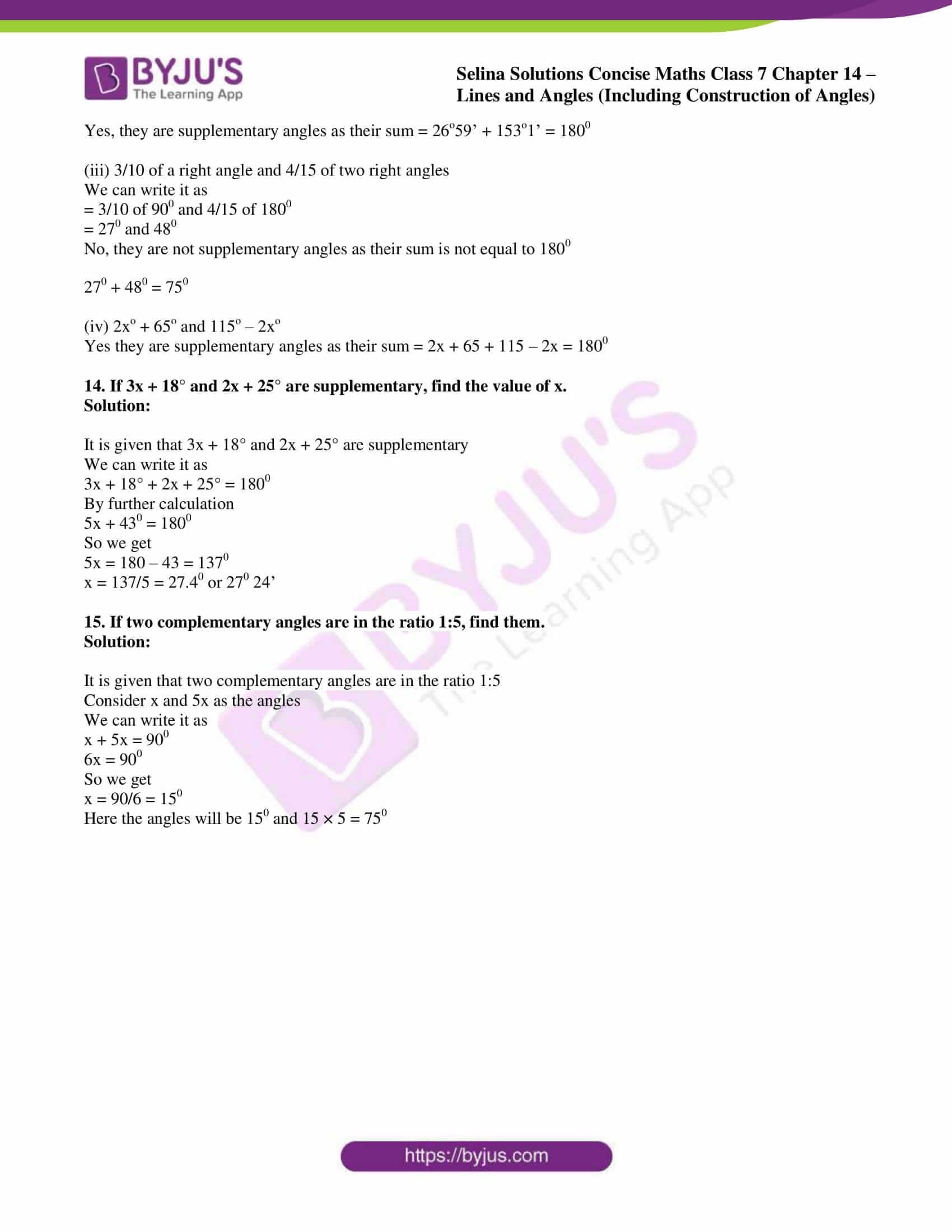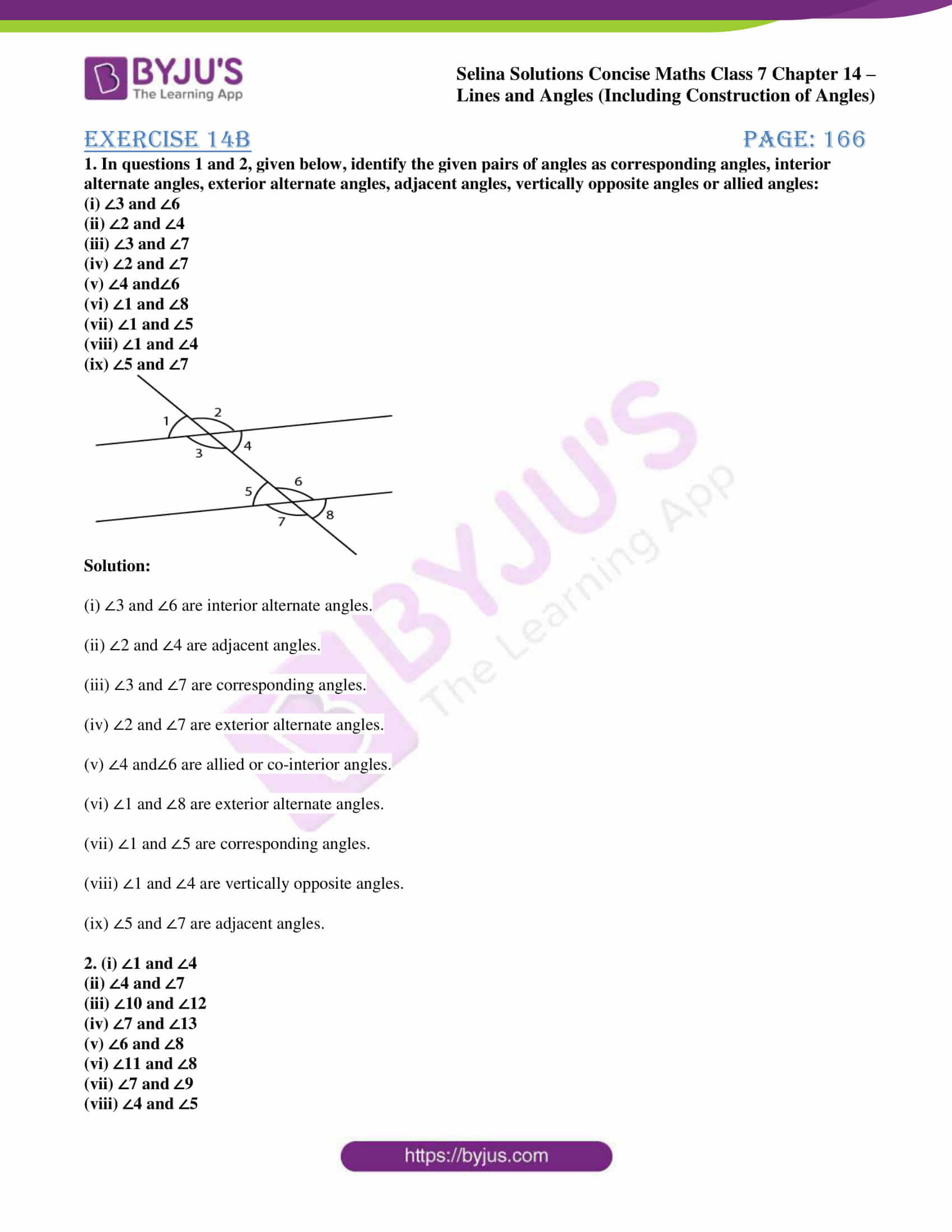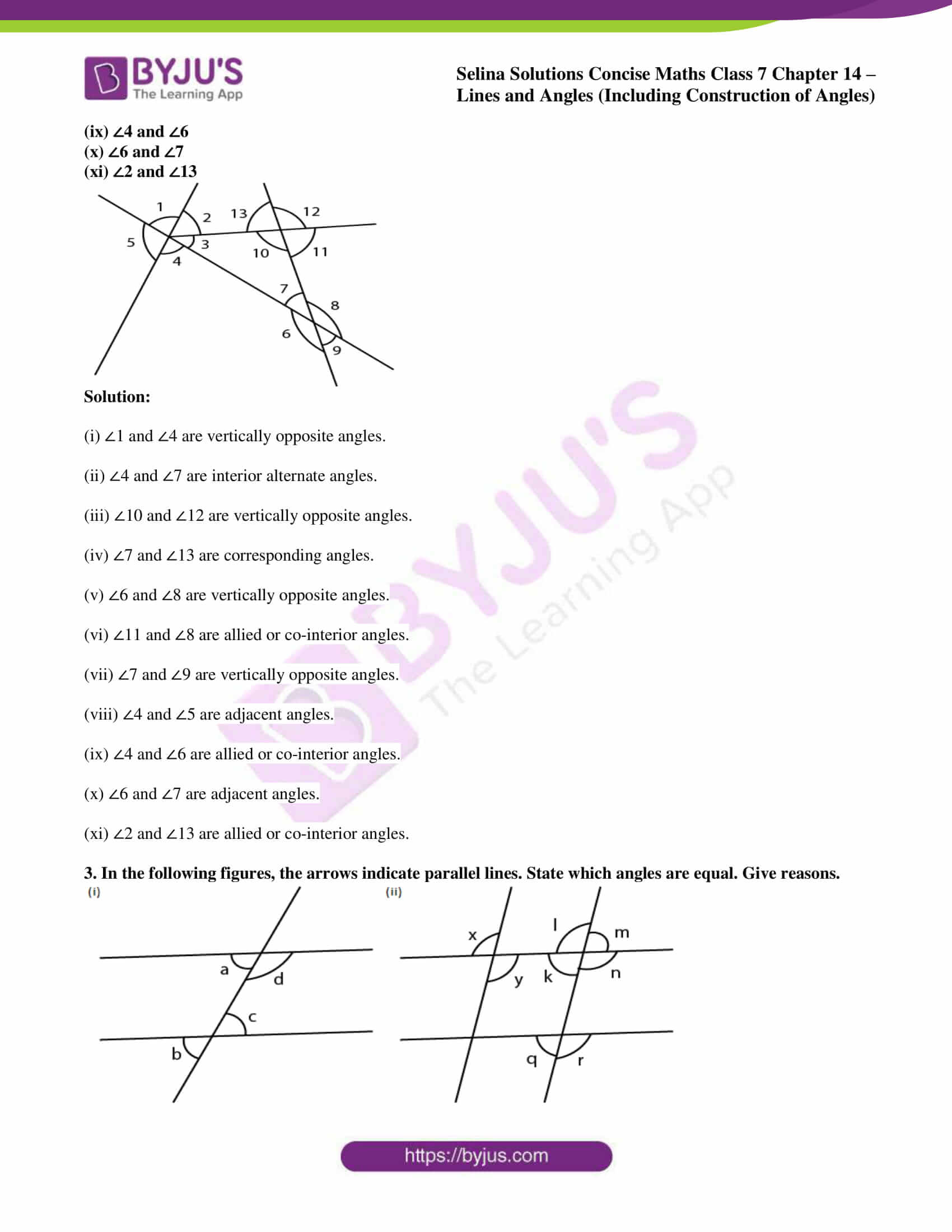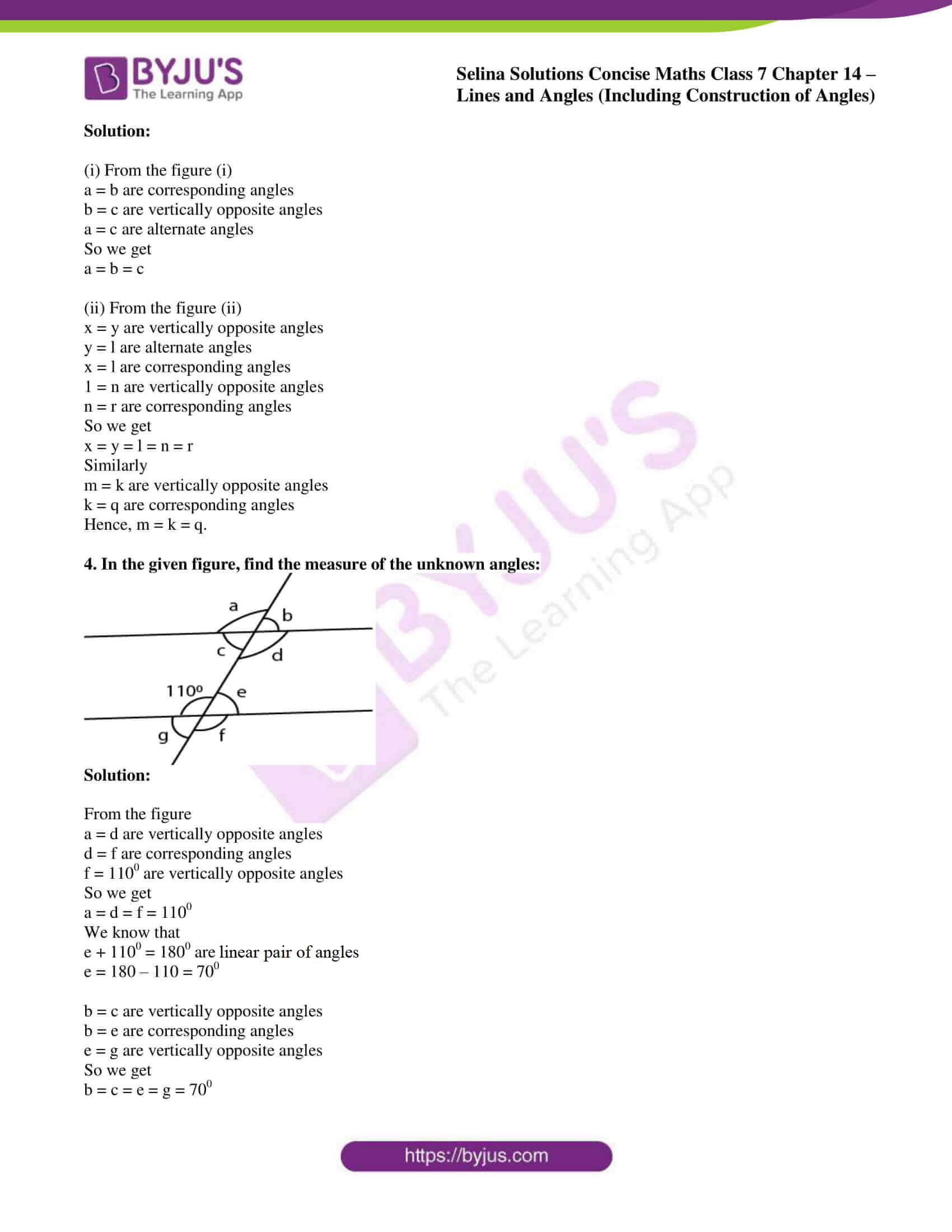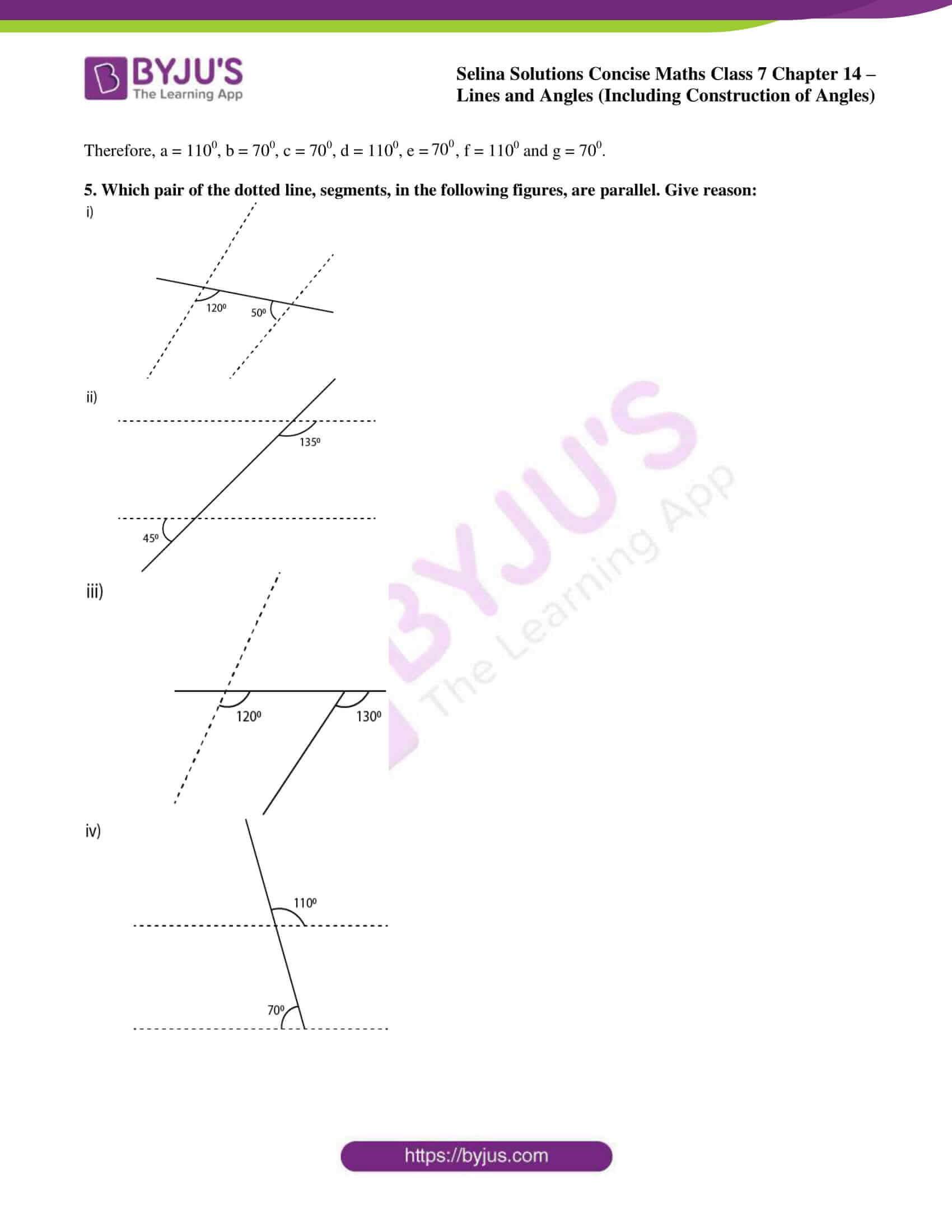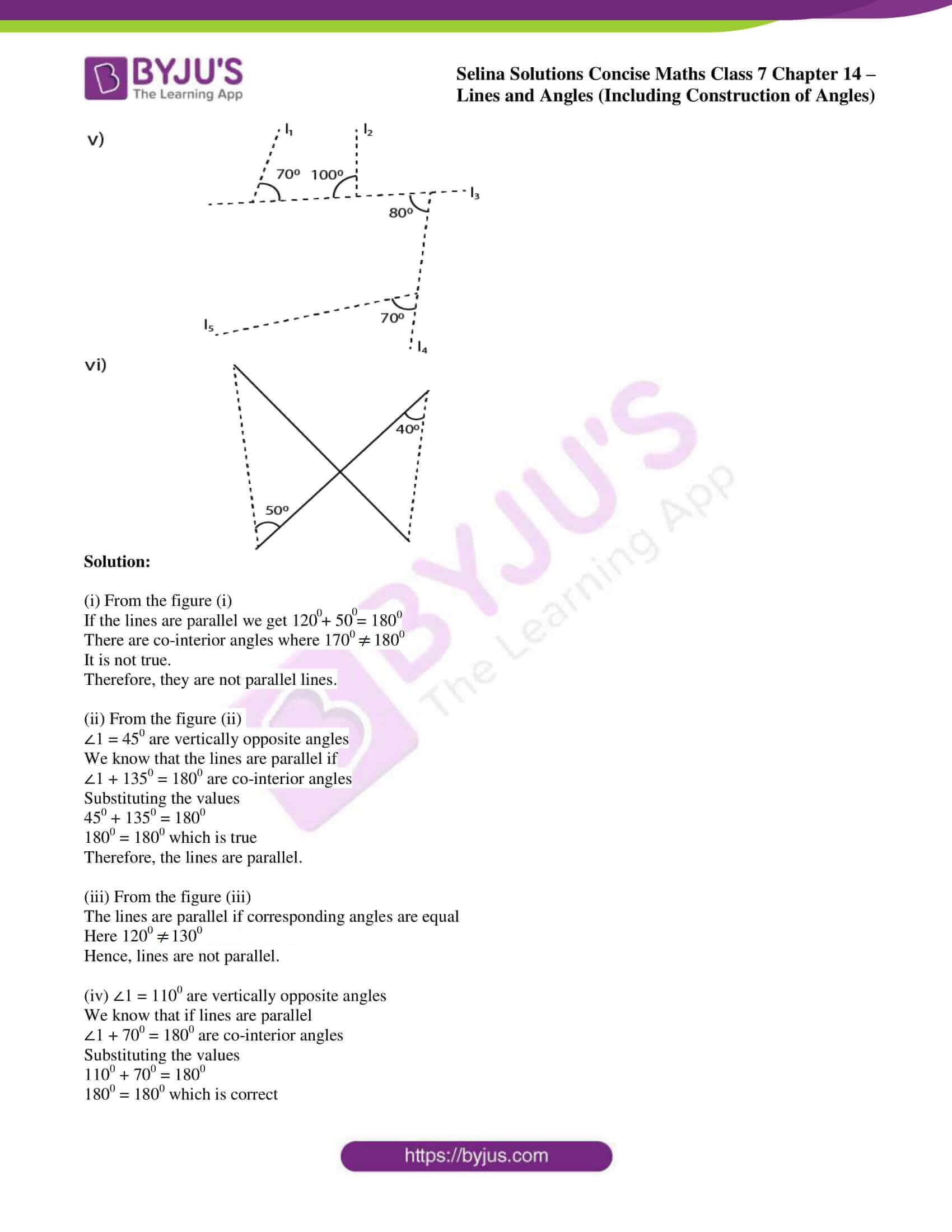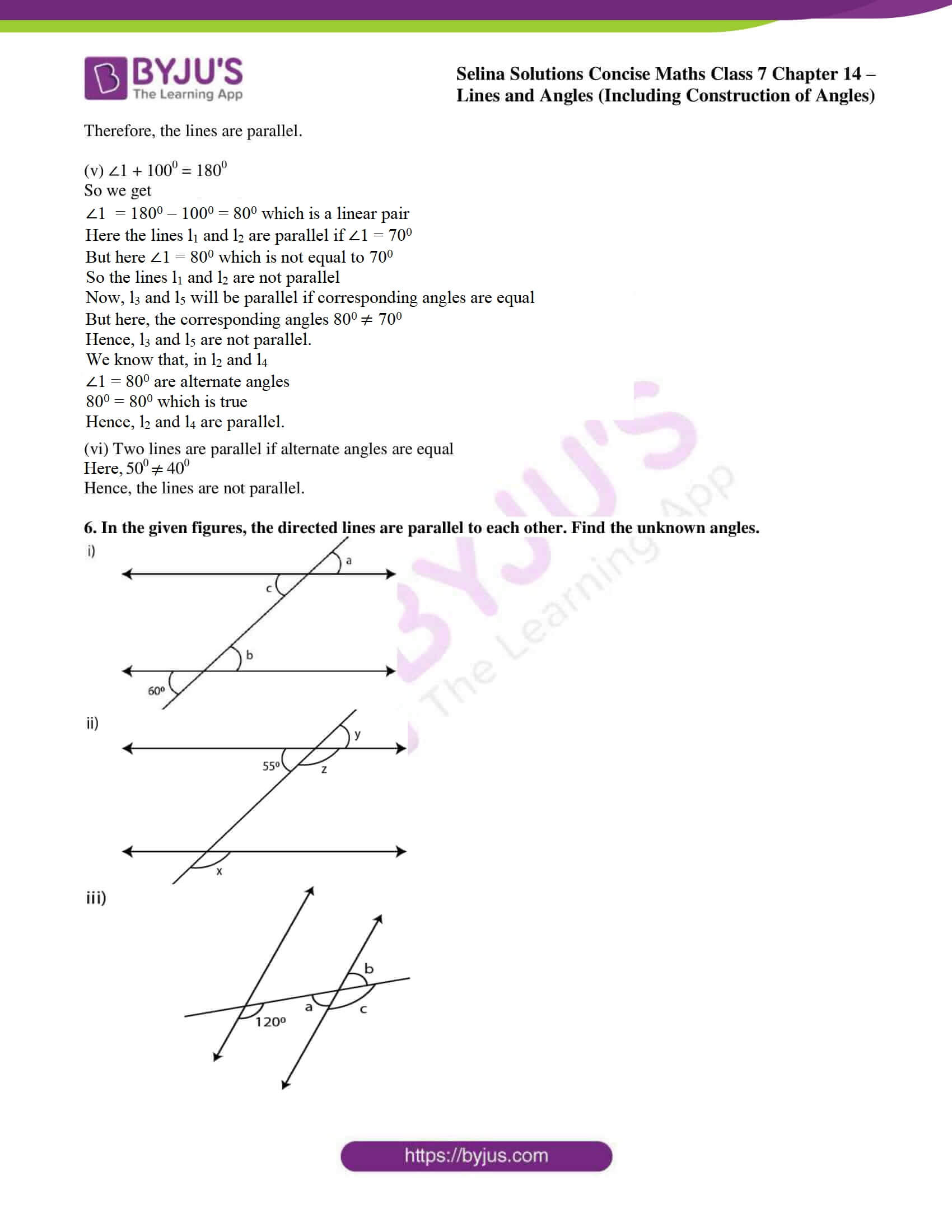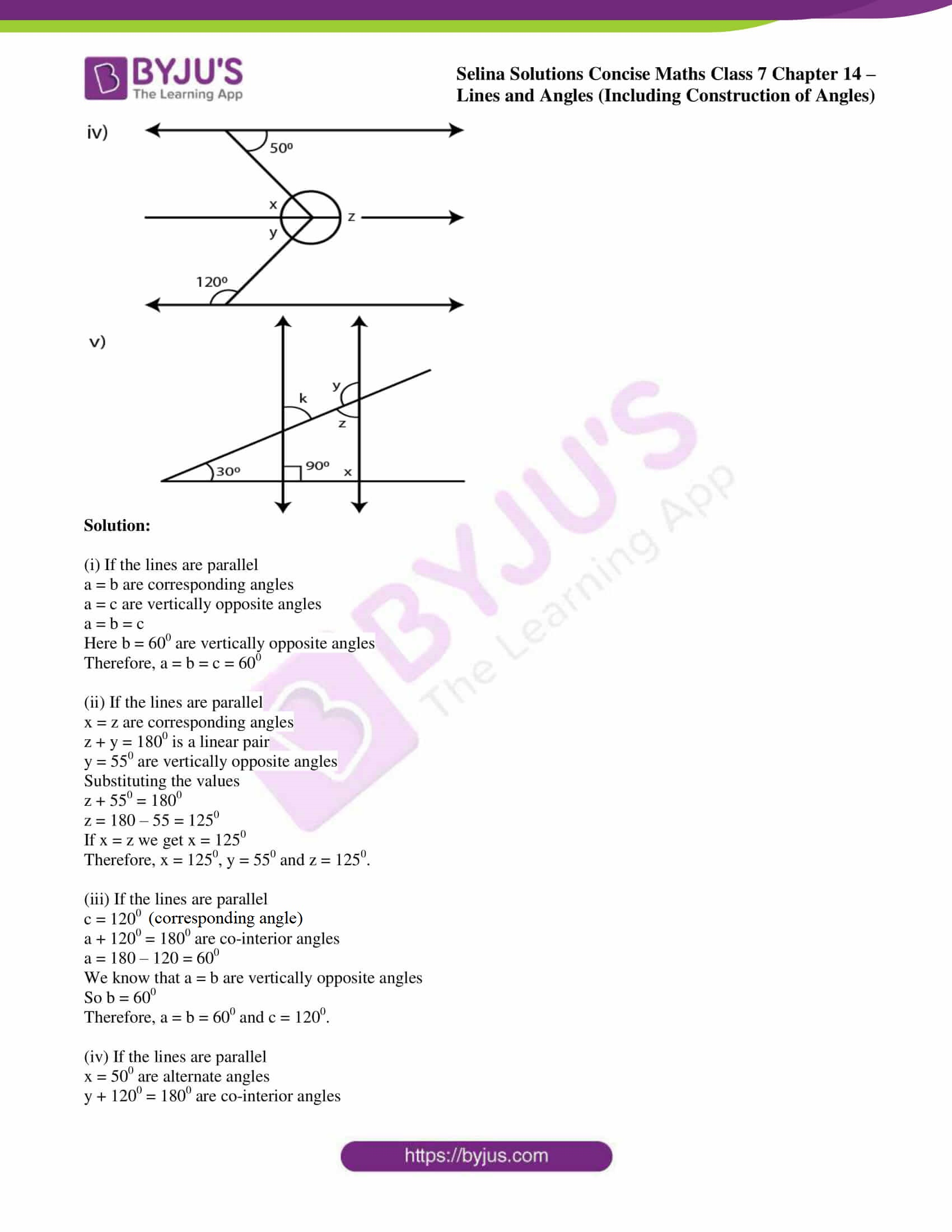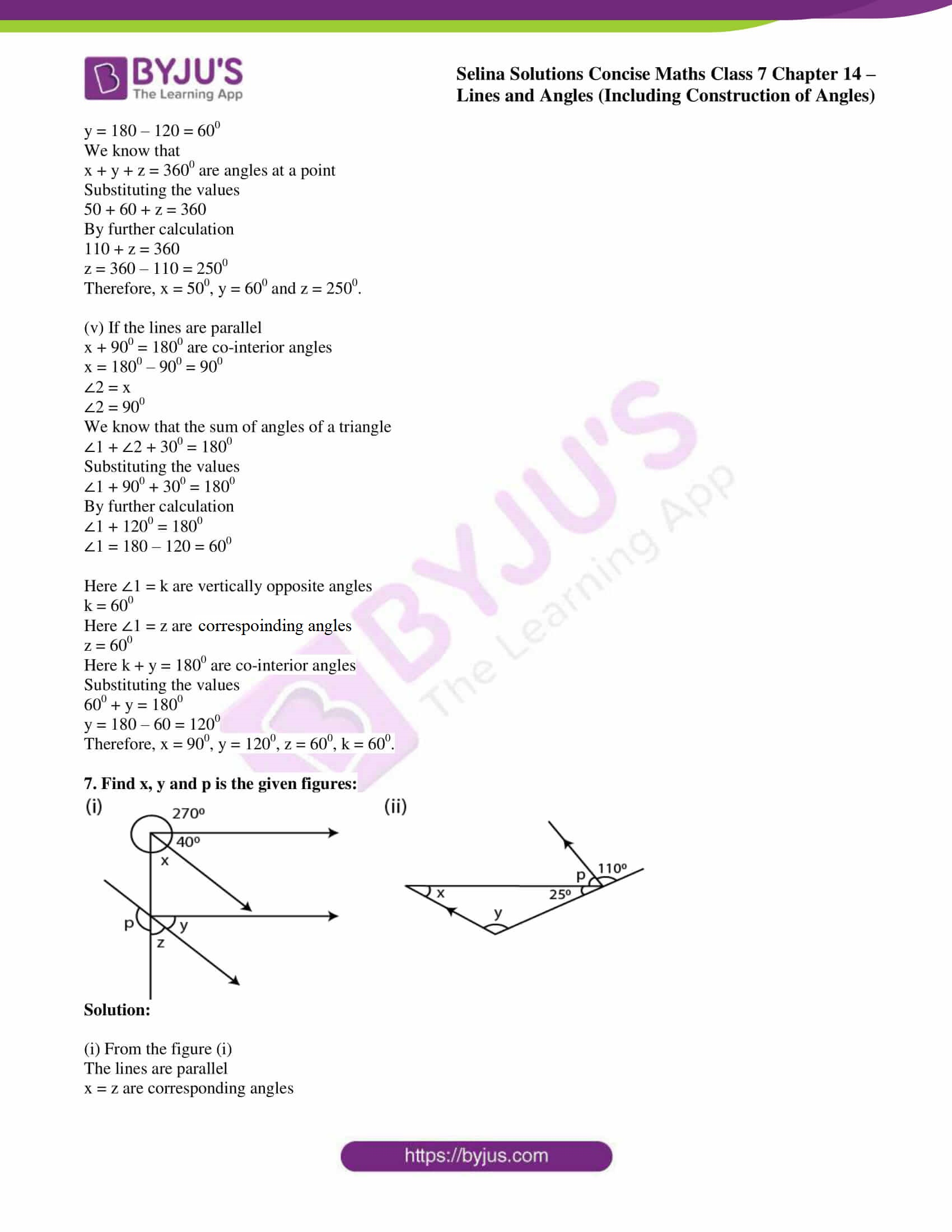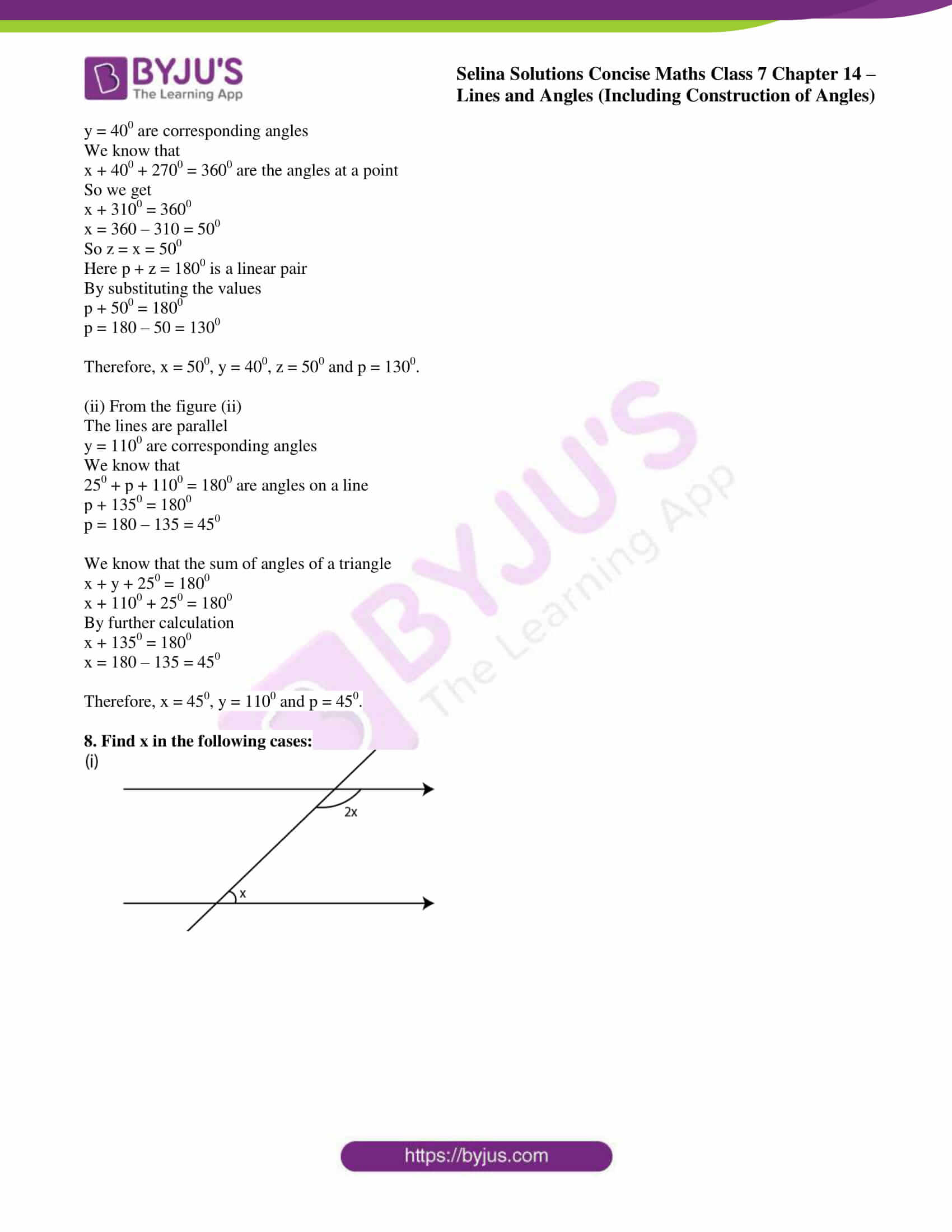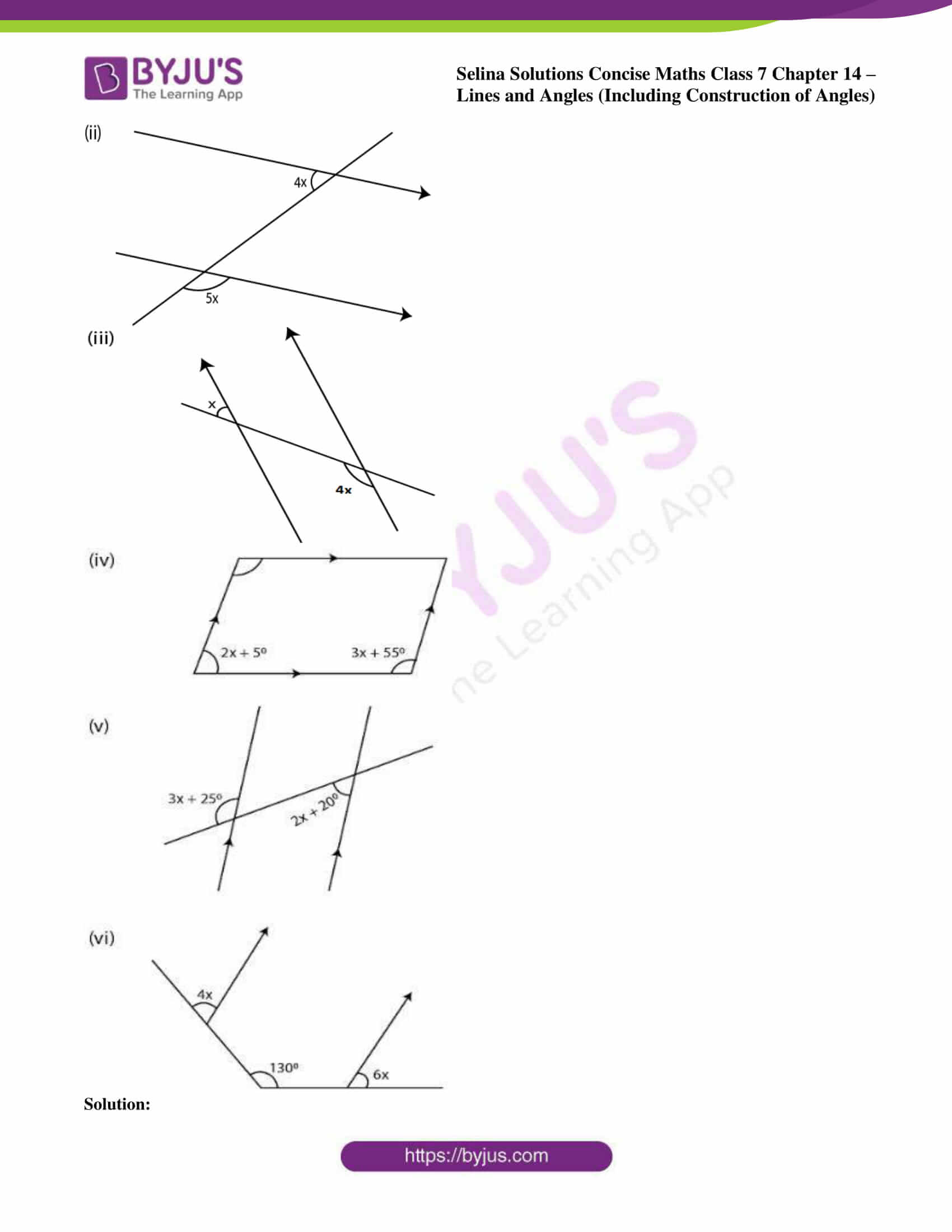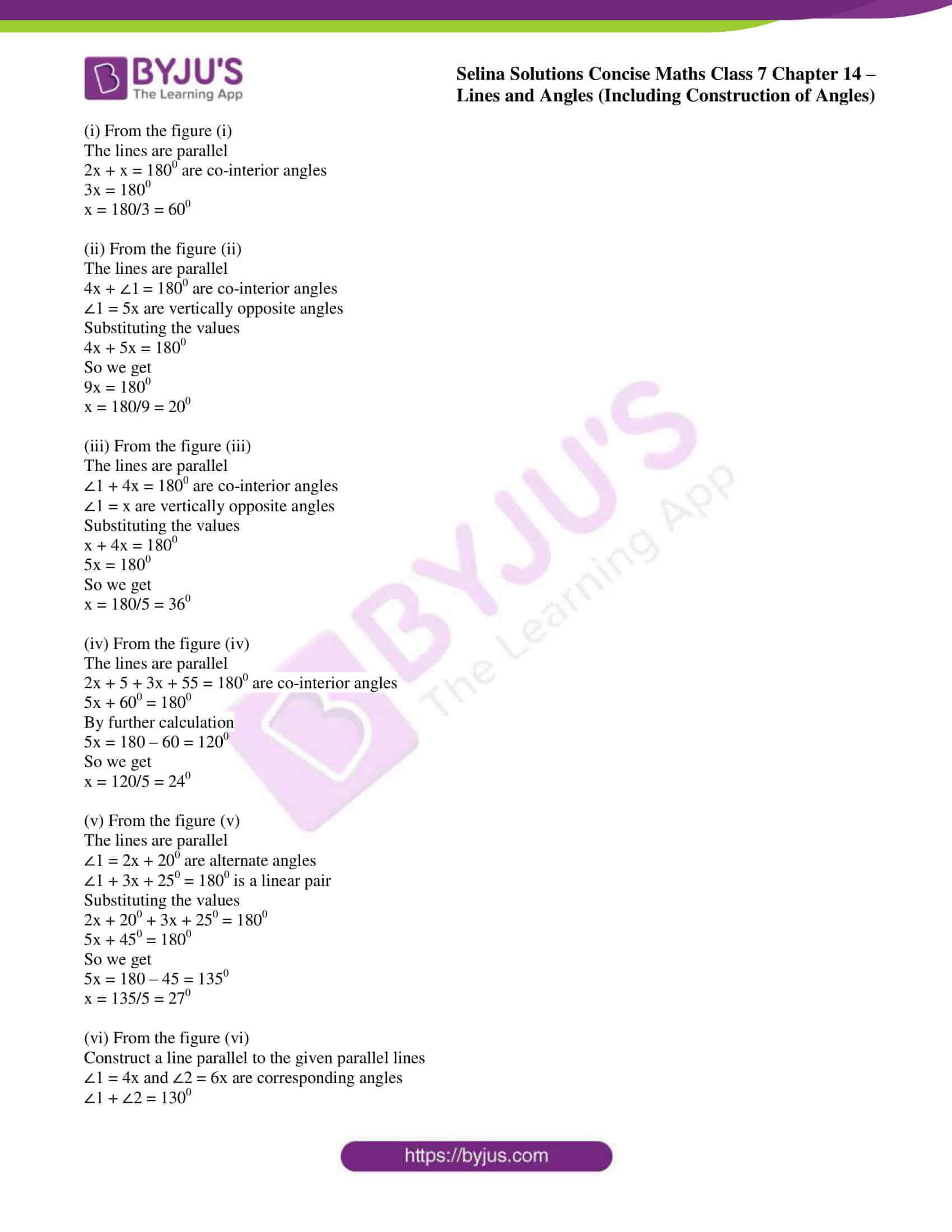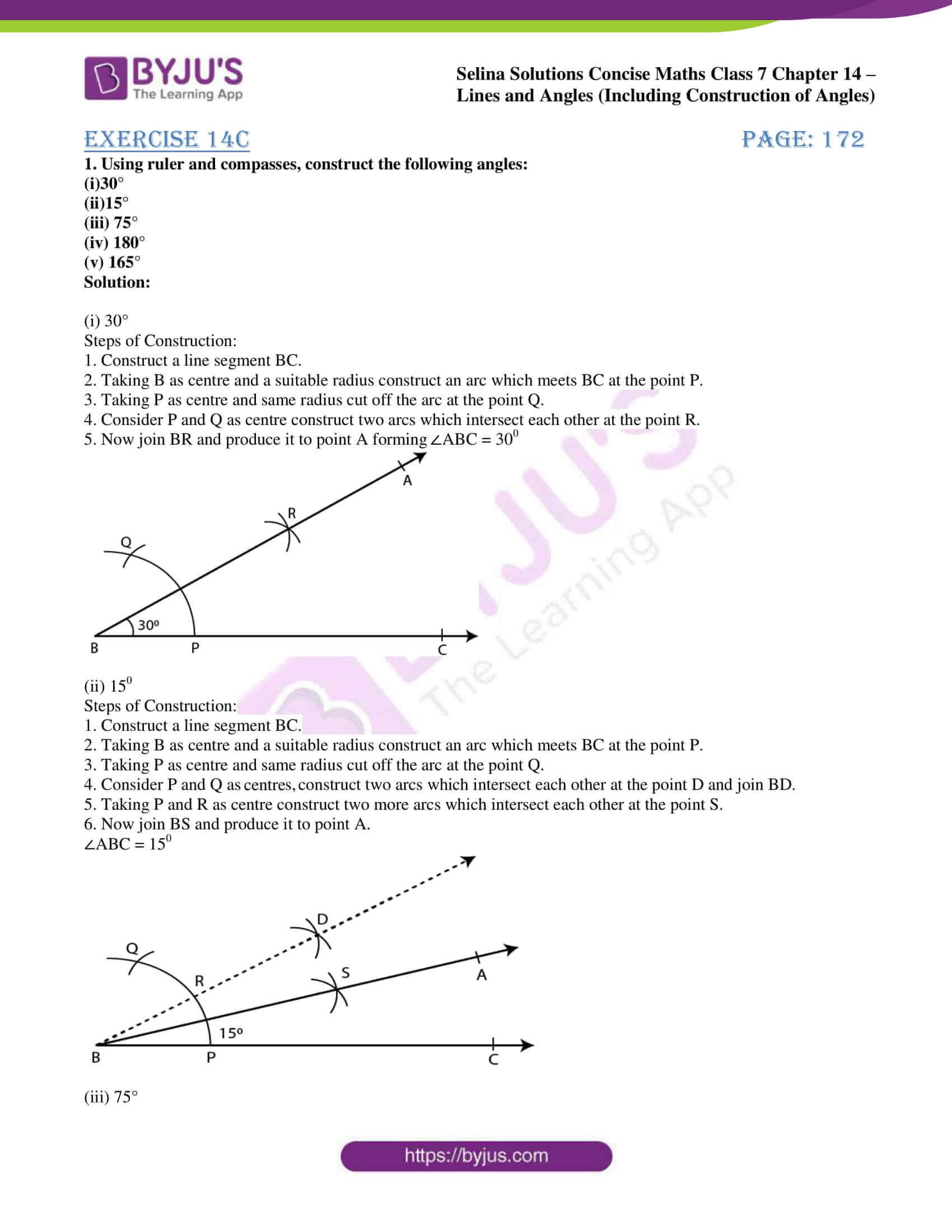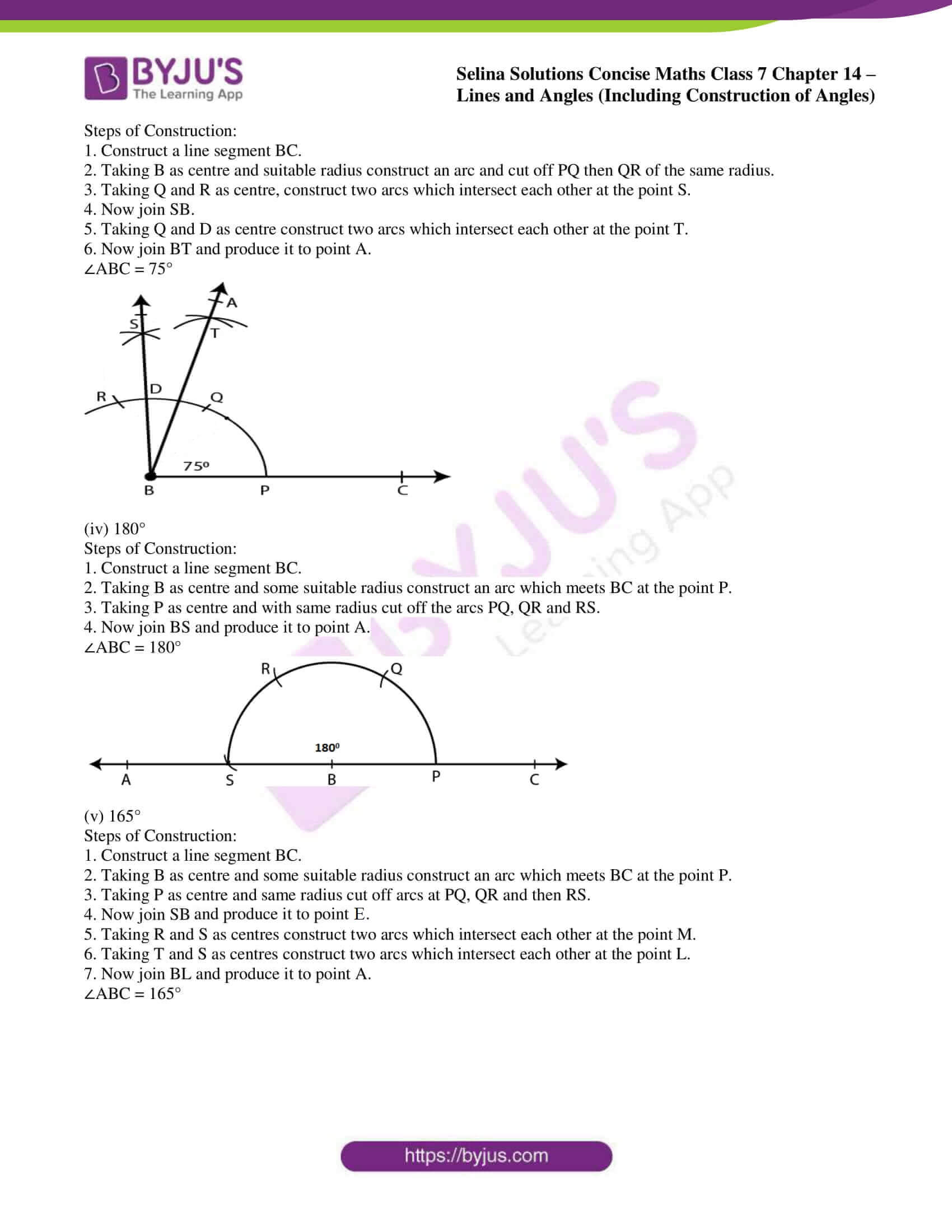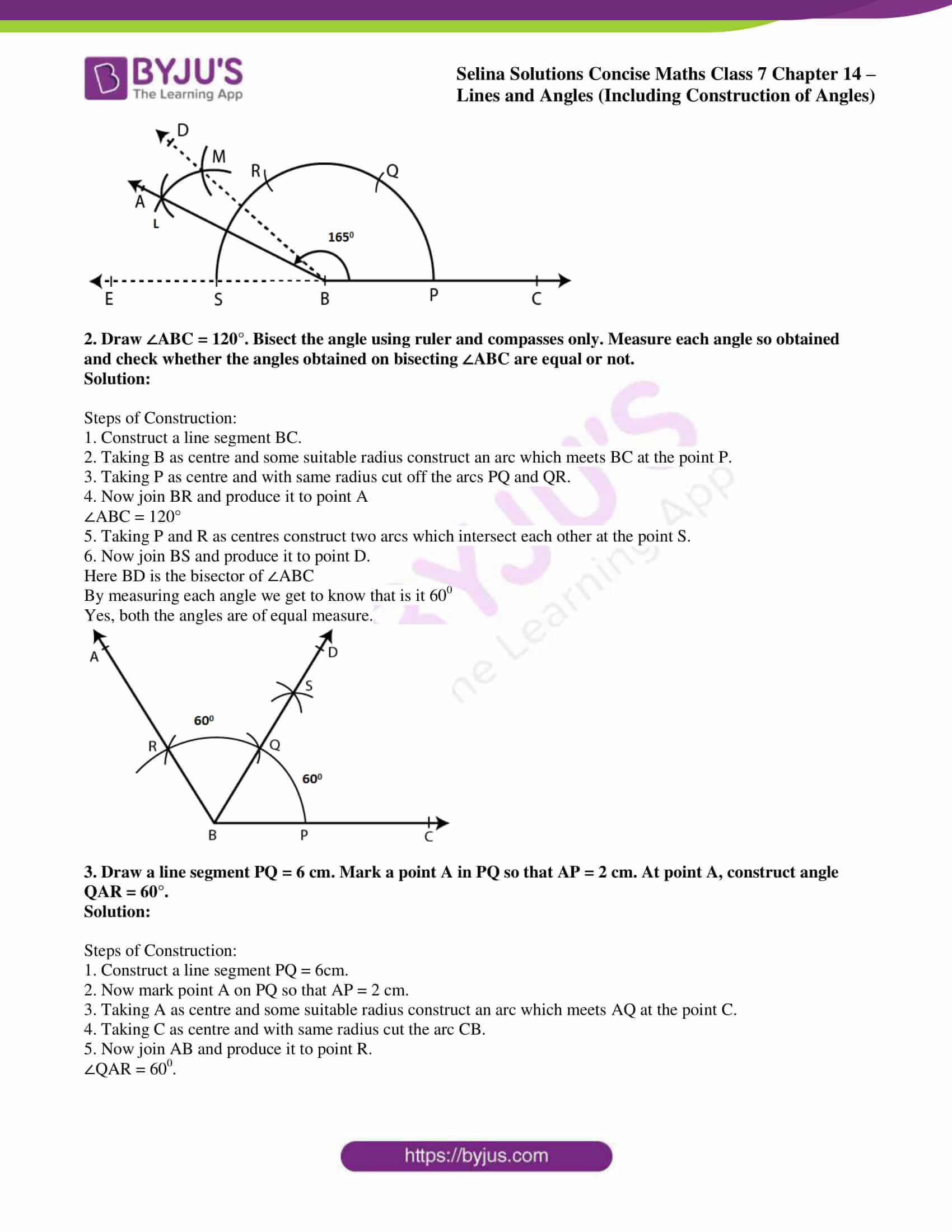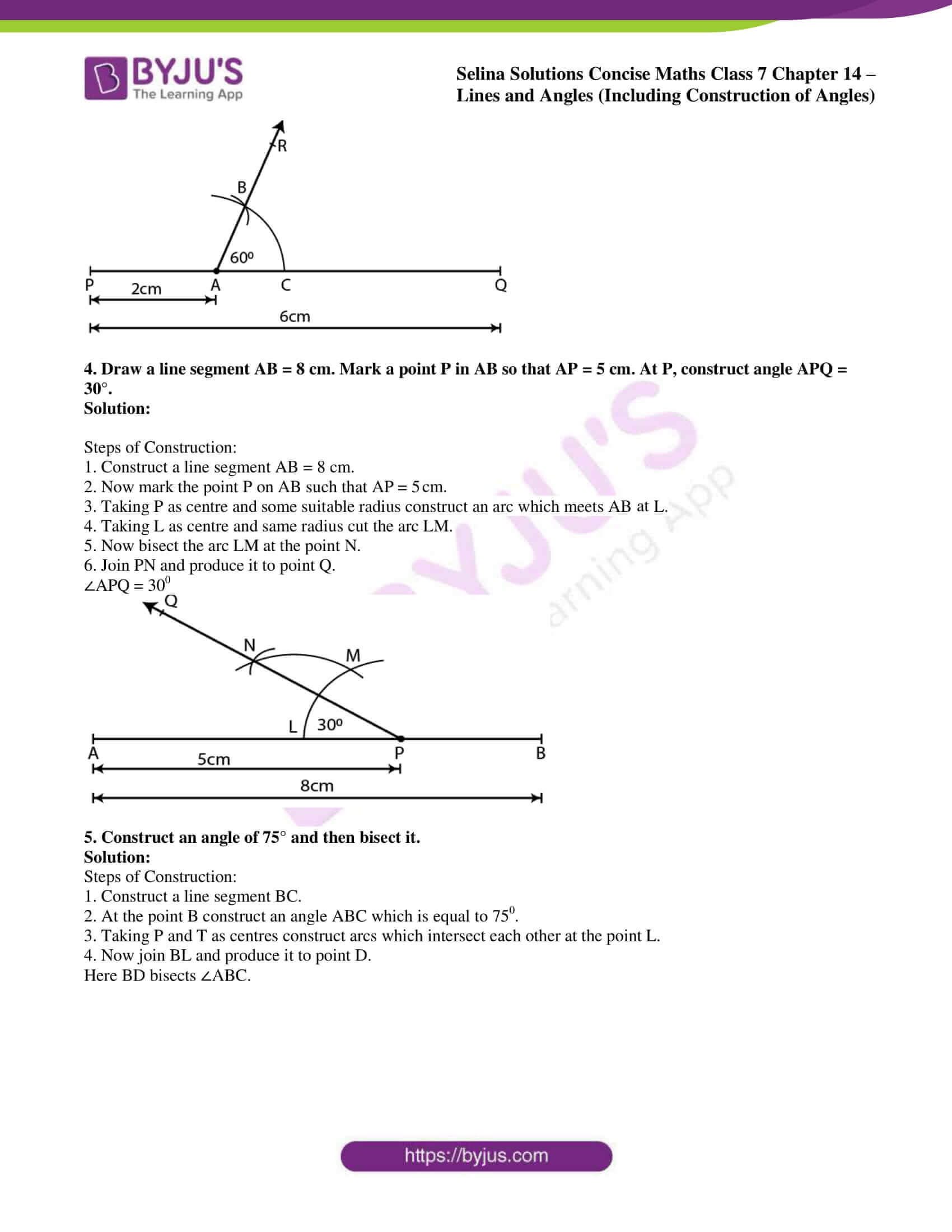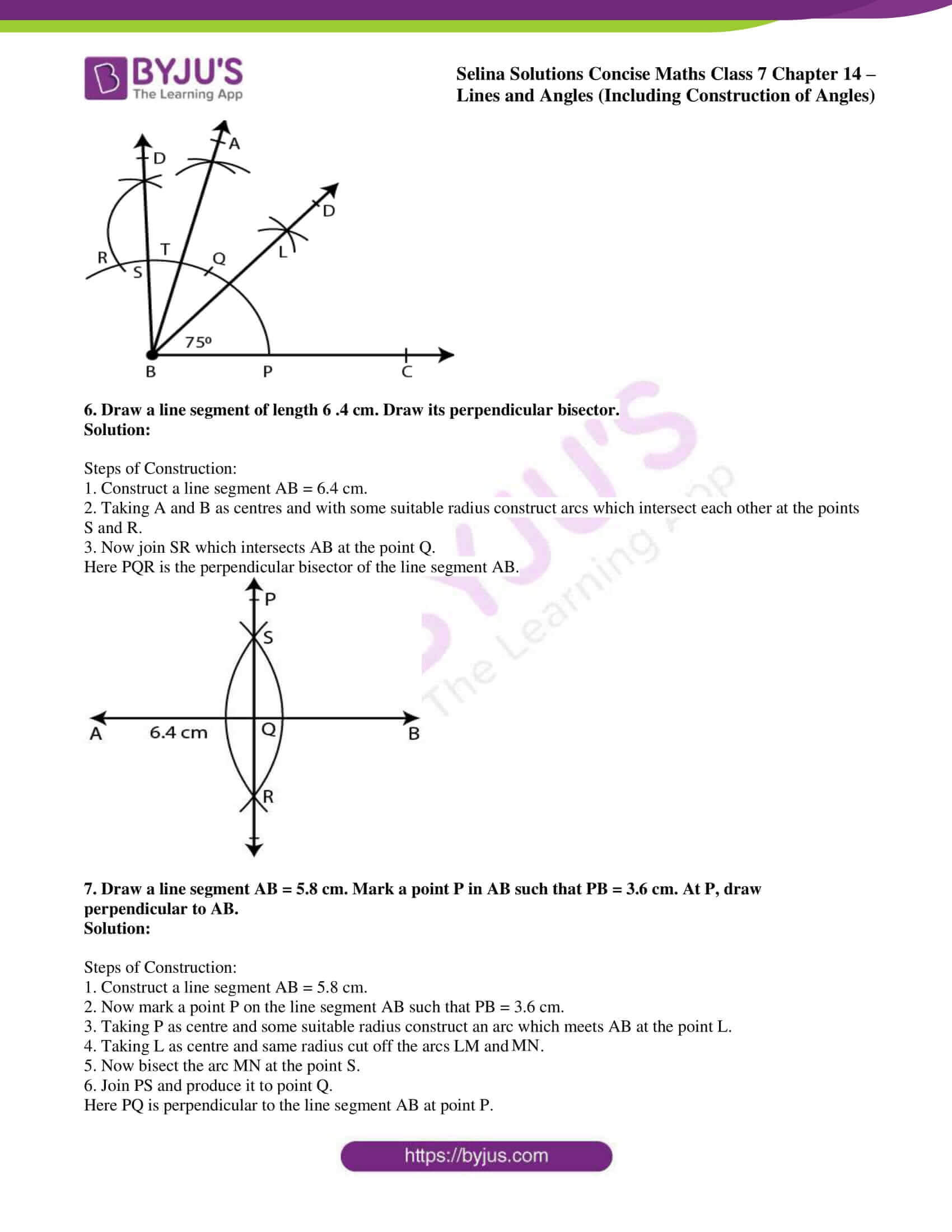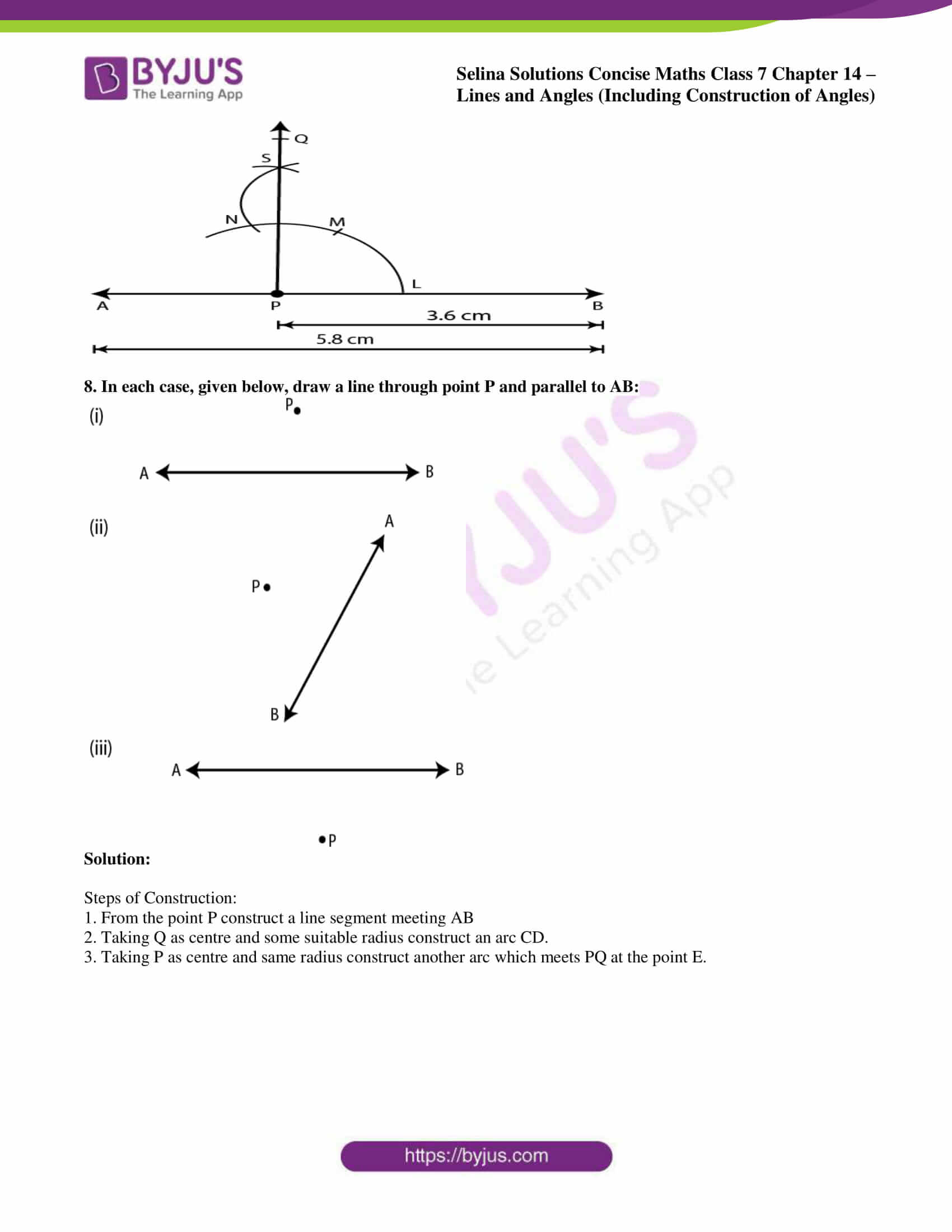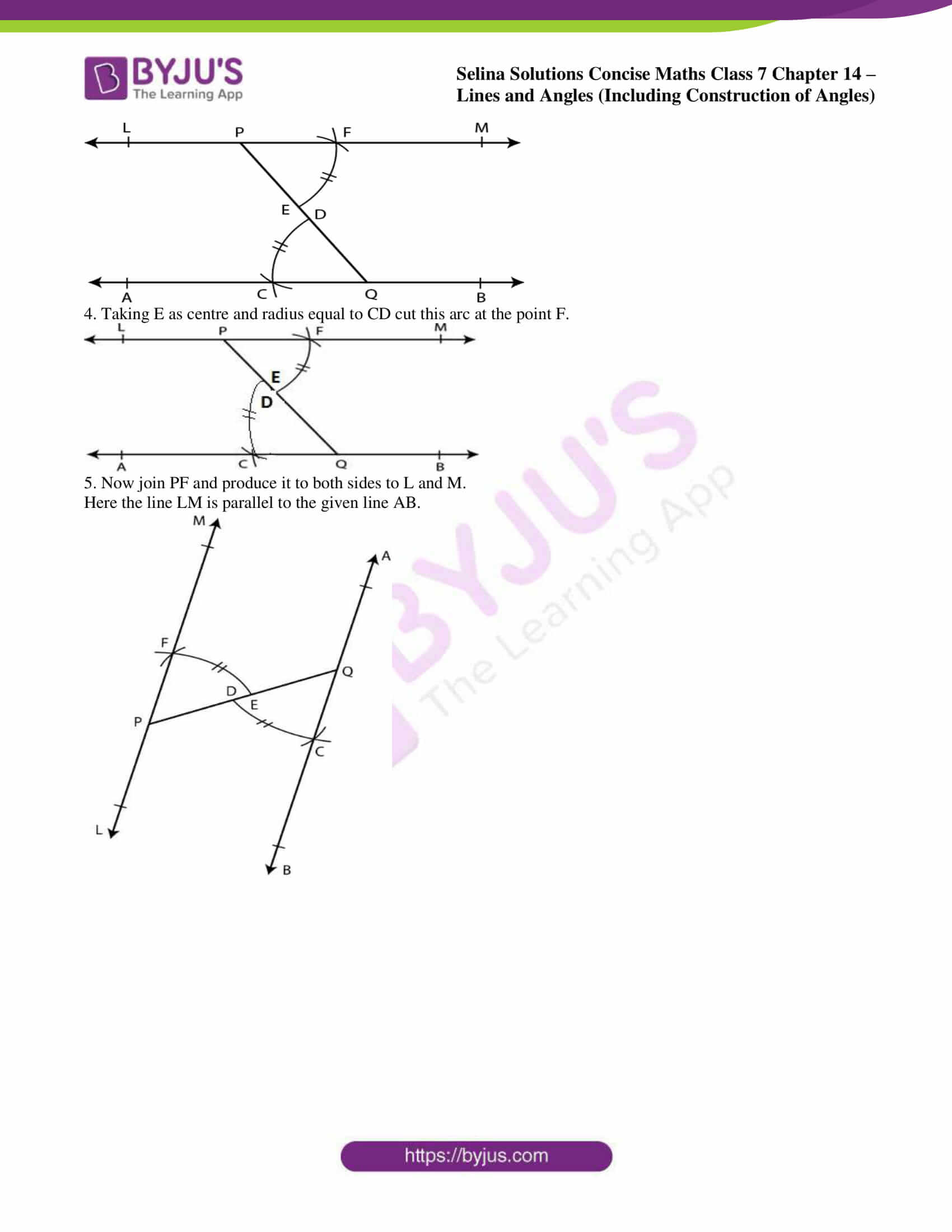### Exercises of Selina Solutions Concise Maths Class 7 Chapter 14 – Lines and Angles (Including Construction of Angles)

Exercise 14A Solutions

Exercise 14B Solutions

Exercise 14C Solutions

## Access Selina Solutions Concise Maths Class 7 Chapter 14: Lines and Angles (Including Construction of Angles)

#### Exercise 14A page: 162

1. State, true or false:

(i) A line segment 4 cm long can have only 2000 points in it.
(ii) A ray has one end point and a line segment has two end-points.
(iii) A line segment is the shortest distance between any two given points.
(iv) An infinite number of straight lines can be drawn through a given point.
(v) Write the number of end points in
(a) a line segment AB (b) a ray AB (c) a line AB
(vi) Out of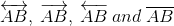which one has a fixed length?
(vii) How many rays can be drawn through a fixed point O?
(viii) How many lines can be drawn through three
(a) collinear points?
(b) non-collinear points?
(ix) Is 40° the complement of 60°?
(x) Is 45° the supplement of 45°?

Solution:

(i) False.

It contains infinite number of points.

(ii) True.

(iii) True.

(iv) True.

(v) (a) 2 (b) 1 (c) 0

(vi)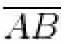has fixed length.

(vii) Infinite rays can be drawn through a fixed point O.

(viii) (a) 1 line can be drawn through three collinear points.

(b) 3 lines can be drawn through three non-collinear points.

(ix) False.

40o is the complement of 50o as 40o + 50o = 90o

(x) False.

45o is the supplement of 135o not 45o.

2. In which of the following figures, are ∠AOB and ∠AOC adjacent angles? Give, in each case, reason for your answer.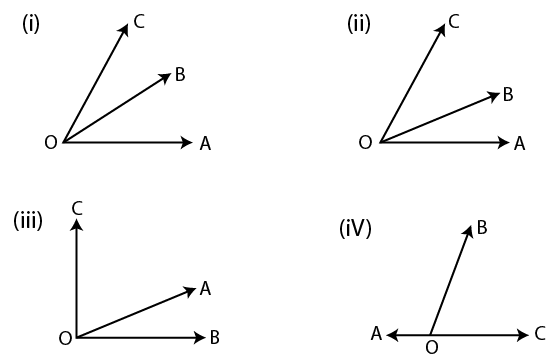Solution:

If ∠AOB and ∠AOC are adjacent angle, they have OA as their common arm.

(i) From the figure

OB is the common arm

∠AOB and ∠AOC are not adjacent angles.

(ii) From the figure

OC is the common arm

∠AOB and ∠AOC are not adjacent angles.

(iii) From the figure

OA is the common arm

∠AOB and ∠AOC are adjacent angles.

(iv) From the figure

OB is the common arm

∠AOB and ∠AOC are not adjacent angles.

3. In the given figure, AOC is a straight line.
Find: (i) x (ii) ∠AOB (iii) ∠BOC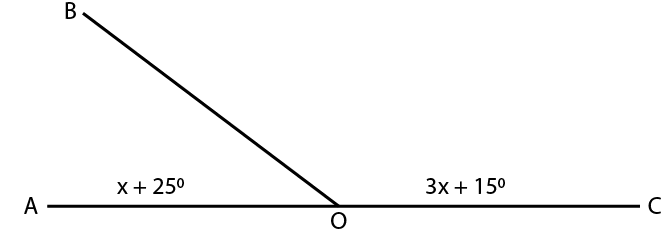Solution:

We know that

∠AOB and ∠COB are linear pairs

It can be written as

∠AOB + ∠COB = 180o

Substituting the values

x + 25o + 3x + 15o = 180o

By further calculation

4x + 40o = 180o

So we get

4x = 180 – 40 = 140o

(i) x = 140/4 = 35o

(ii) ∠AOB = x + 25

Substituting the value of x

∠AOB = 25 + 35 = 60o

(iii) ∠BOC = 3x + 15o

Substituting the value of x

∠BOC = (3 × 35) + 15

∠BOC = 120o

4. Find y in the given figure.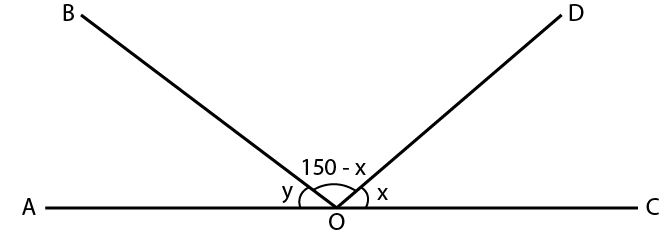Solution:

Here AOC is a straight line

We can write it as

∠AOB + ∠BOD + ∠DOC = 180o

Substituting the values

y + 150 – x + x = 180

By further calculation

y + 150 = 180

So we get

y = 180 – 150 = 30o

5. In the given figure, find ∠PQR.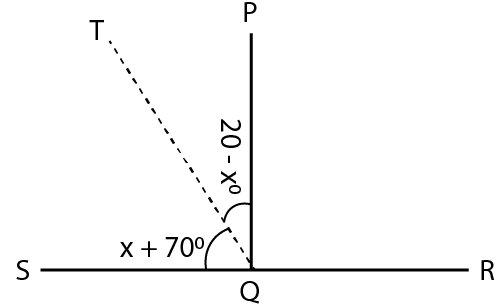Solution:

Here SQR is a straight line

We can write it as

∠SQT + ∠TQP + ∠PQR = 180o

Substituting the values

x + 70 + 20 – x + ∠PQR = 180o

By further calculation

90o + ∠PQR = 180o

So we get

∠PQR = 180o – 90o = 90o

6. In the given figure, po = qo = ro, find each.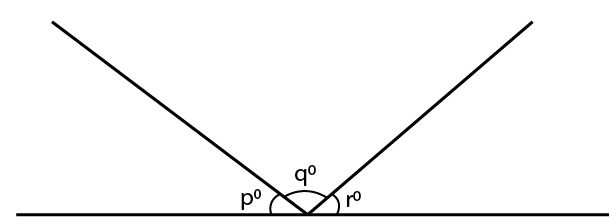Solution:

We know that

po + qo + ro = 180o is a straight angle

It is given that

po = qo = ro

We can write it as

po + po + po = 180o

3p = 180

p = 180/3 = 60o

Therefore, po = qo = ro = 60o

7. In the given figure, if x = 2y, find x and y.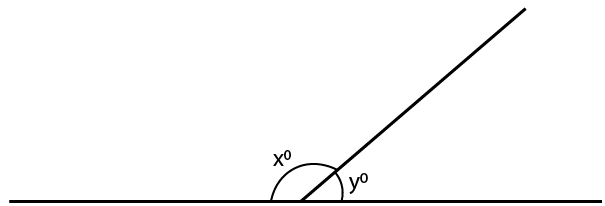Solution:

It is given that

x = 2y

For a straight angle

xo + yo = 180o

Substituting the values

2y + y = 180

By further calculation

3y = 180

y = 180/3 = 60o

x = 2y = 2 × 60o = 120o

8. In the adjoining figure, if bo = ao + co, find b.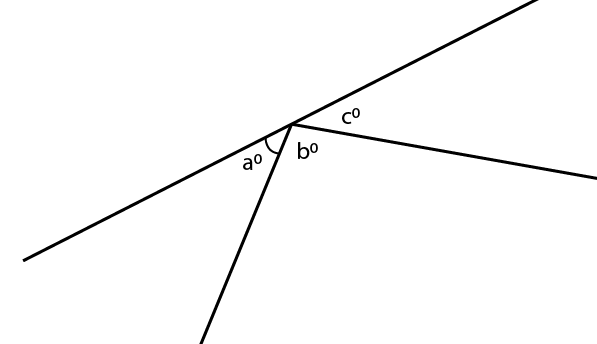Solution:

It is given that

bo = ao + co

For a straight angle

ao + bo + co = 180o

Substituting the values

bo + bo = 180o

2bo = 180o

bo = 180/2 = 90o

9. In the given figure, AB is perpendicular to BC at B.
Find : (i) the value of x.
(ii) the complement of angle x.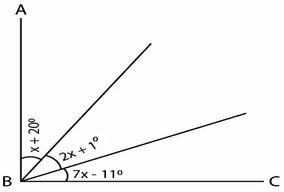Solution:

(i) From the figure

AB || BC at B

Here ∠ABC = 90o

Substituting the values

x + 20 + 2x + 1 + 7x – 11 = 90

By further calculation

10x + 10 = 90

10x = 90 – 10 = 80

x = 80/10 = 8o

(ii) The complement of angle x = 90 – x

So we get

= 90 – 8 = 82o

10. Write the complement of:

(i) 25o

(ii) 90o

(iii) ao

(iv) (x + 5)o

(v) (30 – a)o

(vi) ½ of a right angle

(vii) 1/3 of 180o

(viii) 21o 17’

Solution:

(i) The complement of 25o = 90o – 25o = 65o

(ii) The complement of 90o = 90o – 90o = 0

(iii) The complement of ao = 90o – ao

(iv) The complement of (x + 5)o = 90o – (x + 5)o

By further calculation

= 90o – x – 5o

= 85o – x

(v) The complement of (30 – a)o = 90o – (30 – a)o

By further calculation

= 90o – 30o + ao

= 60o + ao

(vi) The complement of ½ of a right angle = 90o – ½ of a right angle

So we get

= 90o – ½ × 90o

= 90o – 45o

= 45o

(vii) The complement of 1/3 of 180o = 90o – 1/3 of 180o

By further calculation

= 90o – 60o

= 30o

(viii) The complement of 21o 17’ = 90o – 21o 17’

So we get

= 68o 43’

11. Write the supplement of:

(i) 100°
(ii) 0°
(iii) x°
(iv) (x + 35)°
(v) (90 +a + b)°
(vi) (110 – x – 2y)°
(vii) 1/5 of a right angle
(viii) 80° 49′ 25″

Solution:

(i) The supplement of 100° = 180 – 100 = 800

(ii) The supplement of 0° = 180 – 0 = 180o

(iii) The supplement of x° = 1800 – x0

(iv) The supplement of (x + 35)° = 1800 – (x + 35)0

We can write it as

= 180 – x – 35

= 1450 – x0

(v) The supplement of (90 + a + b)° = 1800 – (90 + a + b)0

We can write it as

= 180 – 90 – a – b

So we get

= 900 – a0 – b0

= (90 – a – b)0

(vi) The supplement of (110 – x – 2y)° = 1800 – (110 – x – 2y)°

We can write it as

= 180 – 110 + x + 2y

= 700 + x0 + 2y0

(vii) The supplement of 1/5 of a right angle = 1800 – 1/5 of a right angle

We can write it as

= 1800 – 1/5 × 900

So we get

= 1800 – 180

= 1620

(viii) The supplement of 80° 49′ 25″ = 1800 – 80° 49′ 25″

We know that 10 = 60’ and 1’ = 60”

So we get

= 990 10’ 35”

12. Are the following pairs of angles complementary?

(i) 10° and 80°
(ii) 37° 28′ and 52° 33′
(iii) (x+ 16)°and (74 – x)°
(iv) 54° and 2/5 of a right angle.

Solution:

(i) 10° and 80°

Yes, they are complementary angles as their sum = 100 + 800 = 900

(ii) 37° 28′ and 52° 33′

No, they are not complementary angles as their sum is not equal to 900

37° 28′ + 52° 33′ = 9001’

(iii) (x+ 16)° and (74 – x)°

Yes, they are complementary angles as their sum = x + 16 + 74 – x = 900

(iv) 54° and 2/5 of a right angle

We can write it as

= 540 and 2/5 × 900

= 540 and 360

Yes, they are complementary angles as their sum = 54 + 36 = 900

13. Are the following pairs of angles supplementary?

(i) 139o and 39o

(ii) 26o59’ and 153o1’

(iii) 3/10 of a right angle and 4/15 of two right angles

(iv) 2xo + 65o and 115o – 2xo

Solution:

(i) 139o and 39o

No, they are not supplementary angles as their sum is not equal to 1800

1390 + 390 = 1780

(ii) 26o59’ and 153o1’

Yes, they are supplementary angles as their sum = 26o59’ + 153o1’ = 1800

(iii) 3/10 of a right angle and 4/15 of two right angles

We can write it as

= 3/10 of 900 and 4/15 of 1800

= 270 and 480

No, they are not supplementary angles as their sum is not equal to 1800

270 + 480 = 750

(iv) 2xo + 65o and 115o – 2xo

Yes they are supplementary angles as their sum = 2x + 65 + 115 – 2x = 1800

14. If 3x + 18° and 2x + 25° are supplementary, find the value of x.

Solution:

It is given that 3x + 18° and 2x + 25° are supplementary

We can write it as

3x + 18° + 2x + 25° = 1800

By further calculation

5x + 430 = 1800

So we get

5x = 180 – 43 = 1370

x = 137/5 = 27.40 or 270 24’

15. If two complementary angles are in the ratio 1:5, find them.

Solution:

It is given that two complementary angles are in the ratio 1:5

Consider x and 5x as the angles

We can write it as

x + 5x = 900

6x = 900

So we get

x = 90/6 = 150

Here the angles will be 150 and 15 × 5 = 750

#### Exercise 14B page: 166

1. In questions 1 and 2, given below, identify the given pairs of angles as corresponding angles, interior alternate angles, exterior alternate angles, adjacent angles, vertically opposite angles or allied angles:

(i) ∠3 and ∠6
(ii) ∠2 and ∠4
(iii) ∠3 and ∠7
(iv) ∠2 and ∠7
(v) ∠4 and∠6
(vi) ∠1 and ∠8
(vii) ∠1 and ∠5
(viii) ∠1 and ∠4
(ix) ∠5 and ∠7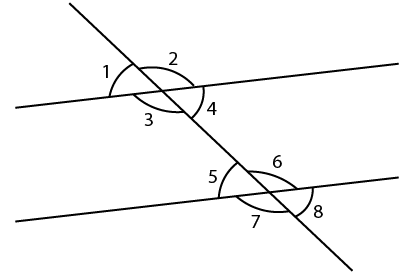Solution:

(i) ∠3 and ∠6 are interior alternate angles.

(ii) ∠2 and ∠4 are adjacent angles.

(iii) ∠3 and ∠7 are corresponding angles.

(iv) ∠2 and ∠7 are exterior alternate angles.

(v) ∠4 and∠6 are allied or co-interior angles.

(vi) ∠1 and ∠8 are exterior alternate angles.

(vii) ∠1 and ∠5 are corresponding angles.

(viii) ∠1 and ∠4 are vertically opposite angles.

(ix) ∠5 and ∠7 are adjacent angles.

2. (i) ∠1 and ∠4
(ii) ∠4 and ∠7
(iii) ∠10 and ∠12
(iv) ∠7 and ∠13
(v) ∠6 and ∠8
(vi) ∠11 and ∠8
(vii) ∠7 and ∠9
(viii) ∠4 and ∠5
(ix) ∠4 and ∠6
(x) ∠6 and ∠7
(xi) ∠2 and ∠13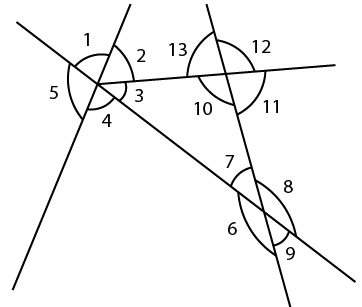Solution:

(i) ∠1 and ∠4 are vertically opposite angles.

(ii) ∠4 and ∠7 are interior alternate angles.

(iii) ∠10 and ∠12 are vertically opposite angles.

(iv) ∠7 and ∠13 are corresponding angles.

(v) ∠6 and ∠8 are vertically opposite angles.

(vi) ∠11 and ∠8 are allied or co-interior angles.

(vii) ∠7 and ∠9 are vertically opposite angles.

(viii) ∠4 and ∠5 are adjacent angles.

(ix) ∠4 and ∠6 are allied or co-interior angles.

(x) ∠6 and ∠7 are adjacent angles.

(xi) ∠2 and ∠13 are allied or co-interior angles.

3. In the following figures, the arrows indicate parallel lines. State which angles are equal. Give reasons.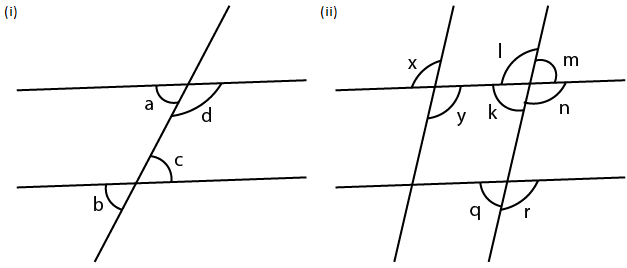Solution:

(i) From the figure (i)

a = b are corresponding angles

b = c are vertically opposite angles

a = c are alternate angles

So we get

a = b = c

(ii) From the figure (ii)

x = y are vertically opposite angles

y = l are alternate angles

x = l are corresponding angles

1 = n are vertically opposite angles

n = r are corresponding angles

So we get

x = y = l = n = r

Similarly

m = k are vertically opposite angles

k = q are corresponding angles

Hence, m = k = q.

4. In the given figure, find the measure of the unknown angles: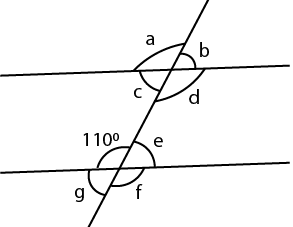Solution:

From the figure

a = d are vertically opposite angles

d = f are corresponding angles

f = 1100 are vertically opposite angles

So we get

a = d = f = 1100

We know that

e + 1100 = 1800 are linear pair of angles

e = 180 – 110 = 700

b = c are vertically opposite angles

b = e are corresponding angles

e = g are vertically opposite angles

So we get

b = c = e = g = 700

Therefore, a = 1100, b = 700, c = 700, d = 1100, e = 700, f = 1100 and g = 700.

5. Which pair of the dotted line, segments, in the following figures, are parallel. Give reason: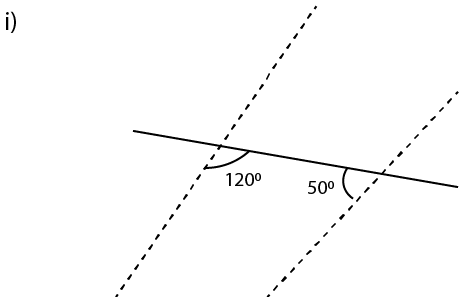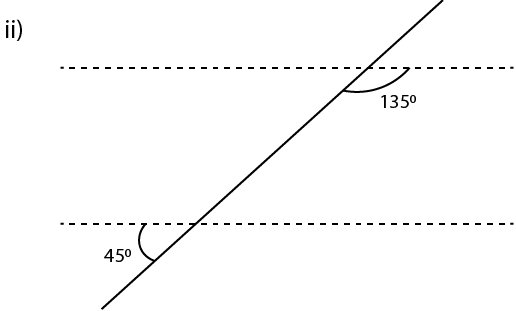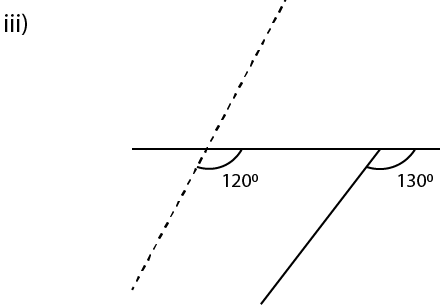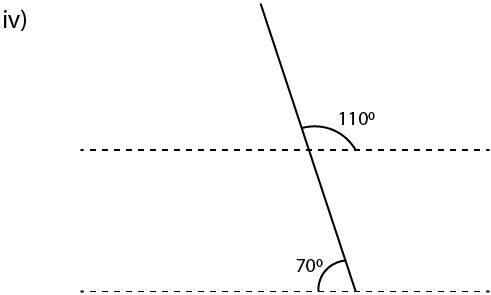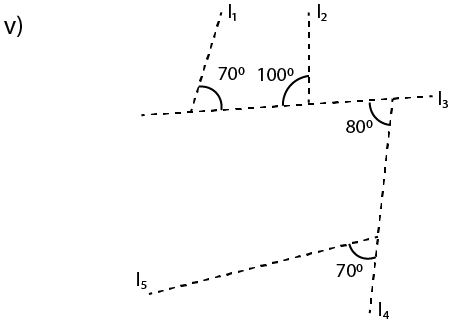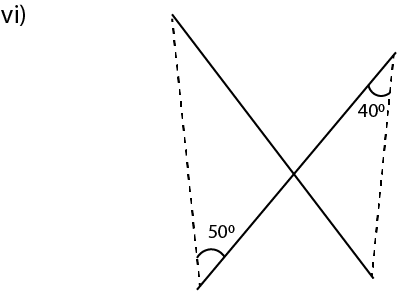Solution:

(i) From the figure (i)

If the lines are parallel we get 1200 + 500 = 1800

There are co-interior angles where 1700 ≠ 1800

Therefore, they are not parallel lines.

(ii) From the figure (ii)

∠1 = 450 are vertically opposite angles

We know that the lines are parallel if

∠1 + 1350 = 1800 are co-interior angles

Substituting the values

450 + 1350 = 1800

1800 = 1800 which is true

Therefore, the lines are parallel.

(iii) From the figure (iii)

The lines are parallel if corresponding angles are equal

Here 1200 ≠ 1300

Hence, lines are not parallel.

(iv) ∠1 = 1100 are vertically opposite angles

We know that if lines are parallel

∠1 + 700 = 1800 are co-interior angles

Substituting the values

1100 + 700 = 1800

1800 = 1800 which is correct

Therefore, the lines are parallel.

(v) ∠1 + 1000 = 1800

So we get

∠1 = 1800 – 1000 = 800 which is a linear pair
Here the lines l1 and l2 are parallel if ∠1 = 700
But here ∠1 = 800 which is not equal to 700
So the lines l1 and l2 are not parallel
Now, l3 and l5 will be parallel if corresponding angles are equal
But here, the corresponding angles 800 ≠ 700
Hence, l3 and l5 are not parallel.
We know that, in l2 and l4
∠1 = 800 are alternate angles
800 = 800 which is true
Hence, l2 and l4 are parallel.

(vi) Two lines are parallel if alternate angles are equal

Here, 500 ≠ 400 which is not true

Hence, the lines are not parallel.

6. In the given figures, the directed lines are parallel to each other. Find the unknown angles.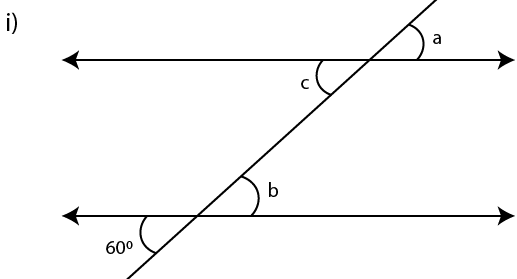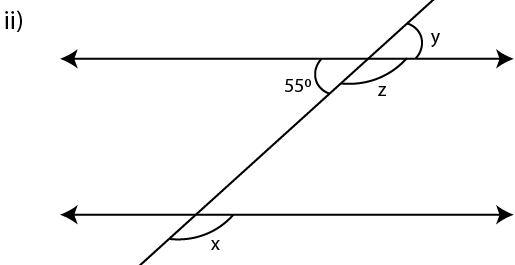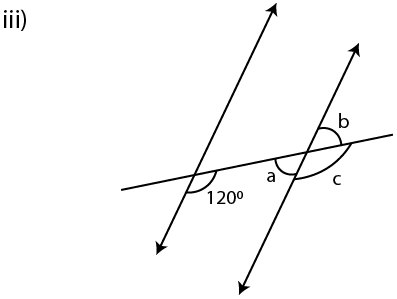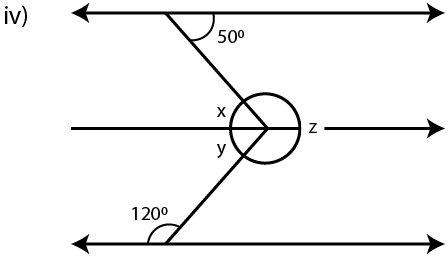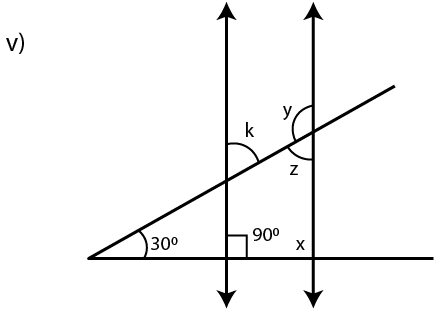Solution:

(i) If the lines are parallel

a = b are corresponding angles

a = c are vertically opposite angles

a = b = c

Here b = 600 are vertically opposite angles

Therefore, a = b = c = 600

(ii) If the lines are parallel

x = z are corresponding angles

z + y = 1800 is a linear pair

y = 550 are vertically opposite angles

Substituting the values

z + 550 = 1800

z = 180 – 55 = 1250

If x = z we get x = 1250

Therefore, x = 1250, y = 550 and z = 1250.

(iii) If the lines are parallel

c = 1200 (corresponding angles)

a + 1200 = 1800 are co-interior angles

a = 180 – 120 = 600

We know that a = b are vertically opposite angles

So b = 600

Therefore, a = b = 600 and c = 1200.

(iv) If the lines are parallel

x = 500 are alternate angles

y + 1200 = 1800 are co-interior angles

y = 180 – 120 = 600

We know that

x + y + z = 3600 are angles at a point

Substituting the values

50 + 60 + z = 360

By further calculation

110 + z = 360

z = 360 – 110 = 2500

Therefore, x = 500, y = 600 and z = 2500.

(v) If the lines are parallel

x + 900 = 1800 are co-interior angles

x = 1800 – 900 = 900

∠2 = x

∠2 = 900

We know that the sum of angles of a triangle

∠1 + ∠2 + 300 = 1800

Substituting the values

∠1 + 900 + 300 = 1800

By further calculation

∠1 + 1200 = 1800

∠1 = 180 – 120 = 600

Here ∠1 = k are vertically opposite angles

k = 600

Here ∠1 = z are corresponding angles

z = 600

Here k + y = 1800 are co-interior angles

Substituting the values

600 + y = 1800

y = 180 – 60 = 1200

Therefore, x = 900, y = 1200, z = 600, k = 600.

7. Find x, y and p is the given figures: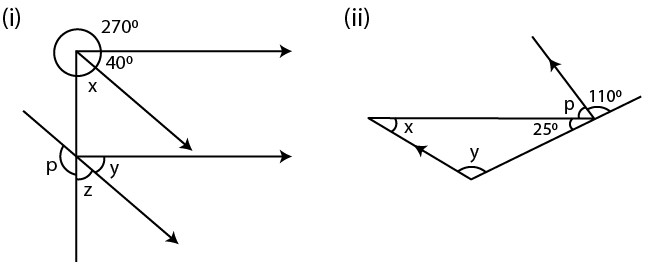Solution:

(i) From the figure (i)

The lines are parallel

x = z are corresponding angles

y = 400 are corresponding angles

We know that

x + 400 + 2700 = 3600 are the angles at a point

So we get

x + 3100 = 3600

x = 360 – 310 = 500

So z = x = 500

Here p + z = 1800 is a linear pair

By substituting the values

p + 500 = 1800

p = 180 – 50 = 1300

Therefore, x = 500, y = 400, z = 500 and p = 1300.

(ii) From the figure (ii)

The lines are parallel

y = 1100 are corresponding angles

We know that

250 + p + 1100 = 1800 are angles on a line

p + 1350 = 1800

p = 180 – 135 = 450

We know that the sum of angles of a triangle

x + y + 250 = 1800

x + 1100 + 250 = 1800

By further calculation

x + 1350 = 1800

x = 180 – 135 = 450

Therefore, x = 450, y = 1100 and p = 450.

8. Find x in the following cases: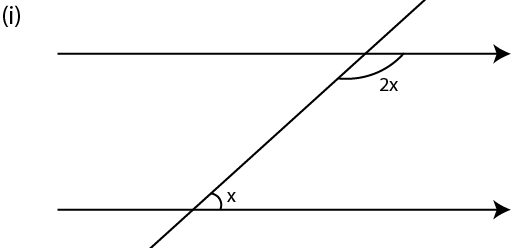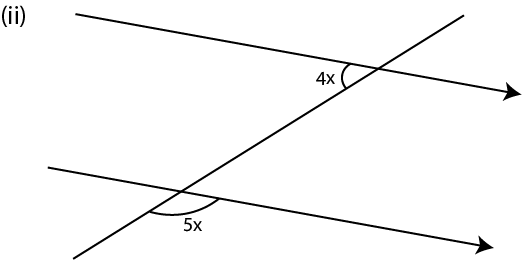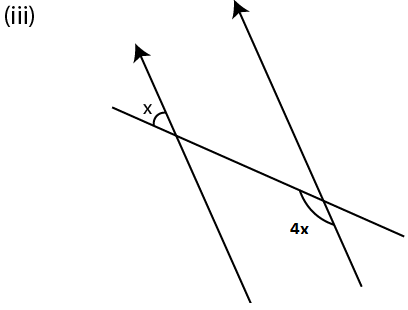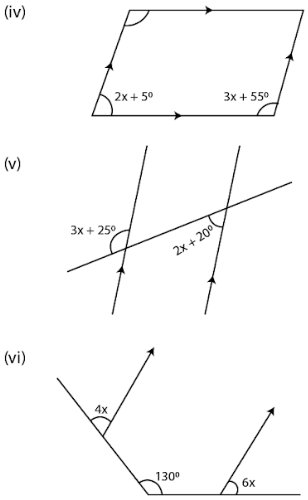Solution:

(i) From the figure (i)

The lines are parallel

2x + x = 1800 are co-interior angles

3x = 1800

x = 180/3 = 600

(ii) From the figure (ii)

The lines are parallel

4x + ∠1 = 1800 are co-interior angles

∠1 = 5x are vertically opposite angles

Substituting the values

4x + 5x = 1800

So we get

9x = 1800

x = 180/9 = 200

(iii) From the figure (iii)

The lines are parallel

∠1 + 4x = 1800 are co-interior angles

∠1 = x are vertically opposite angles

Substituting the values

x + 4x = 1800

5x = 1800

So we get

x = 180/5 = 360

(iv) From the figure (iv)

The lines are parallel

2x + 5 + 3x + 55 = 1800 are co-interior angles

5x + 600 = 1800

By further calculation

5x = 180 – 60 = 1200

So we get

x = 120/5 = 240

(v) From the figure (v)

The lines are parallel

∠1 = 2x + 200 are alternate angles

∠1 + 3x + 250 = 1800 is a linear pair

Substituting the values

2x + 200 + 3x + 250 = 1800

5x + 450 = 1800

So we get

5x = 180 – 45 = 1350

x = 135/5 = 270

(vi) From the figure (vi)

Construct a line parallel to the given parallel lines

∠1 = 4x and ∠2 = 6x are corresponding angles

∠1 + ∠2 = 1300

Substituting the values

4x + 6x = 1300

10x = 1300

So we get

x = 130

e + 1100 = 1800 are linear pair of angles

e = 180 – 110 = 700

b = c are vertically opposite angles

b = e are corresponding angles

e = g are vertically opposite angles

So we get

b = c = e = g = 700

Therefore, a = 1100, b = 700, c = 700, d = 1100, e = 700, f = 1100 and g = 700.

5. Which pair of the dotted line, segments, in the following figures, are parallel. Give reason:Solution:

(i) From the figure (i)

If the lines are parallel we get 1200 + 500 = 1800

There are co-interior angles where 1700 ≠ 1800

Therefore, they are not parallel lines.

(ii) From the figure (ii)

∠1 = 450 are vertically opposite angles

We know that the lines are parallel if

∠1 + 1350 = 1800 are co-interior angles

Substituting the values

450 + 1350 = 1800

1800 = 1800 which is true

Therefore, the lines are parallel.

(iii) From the figure (iii)

The lines are parallel if corresponding angles are equal

Here 1200 ≠ 1300

Hence, lines are not parallel.

(iv) ∠1 = 1100 are vertically opposite angles

We know that if lines are parallel

∠1 + 700 = 1800 are co-interior angles

Substituting the values

1100 + 700 = 1800

1800 = 1800 which is correct

Therefore, the lines are parallel.

(v) ∠1 + 1000 = 1800

So we get

∠1 = 1800 – 1000 = 800 which is a linear pair
Here the lines l1 and l2 are parallel if ∠1 = 700
But here ∠1 = 800 which is not equal to 700
So the lines l1 and l2 are not parallel
Now, l3 and l5 will be parallel if corresponding angles are equal
But here, the corresponding angles 800 ≠ 700
Hence, l3 and l5 are not parallel.
We know that, in l2 and l4
∠1 = 800 are alternate angles
800 = 800 which is true
Hence, l2 and l4 are parallel.

(vi) Two lines are parallel if alternate angles are equal

Here, 500 ≠ 400 which is not true

Hence, the lines are not parallel.

6. In the given figures, the directed lines are parallel to each other. Find the unknown angles.Solution:

(i) If the lines are parallel

a = b are corresponding angles

a = c are vertically opposite angles

a = b = c

Here b = 600 are vertically opposite angles

Therefore, a = b = c = 600

(ii) If the lines are parallel

x = z are corresponding angles

z + y = 1800 is a linear pair

y = 550 are vertically opposite angles

Substituting the values

z + 550 = 1800

z = 180 – 55 = 1250

If x = z we get x = 1250

Therefore, x = 1250, y = 550 and z = 1250.

(iii) If the lines are parallel

c = 1200 (corresponding angles)

a + 1200 = 1800 are co-interior angles

a = 180 – 120 = 600

We know that a = b are vertically opposite angles

So b = 600

Therefore, a = b = 600 and c = 1200.

(iv) If the lines are parallel

x = 500 are alternate angles

y + 1200 = 1800 are co-interior angles

y = 180 – 120 = 600

We know that

x + y + z = 3600 are angles at a point

Substituting the values

50 + 60 + z = 360

By further calculation

110 + z = 360

z = 360 – 110 = 2500

Therefore, x = 500, y = 600 and z = 2500.

(v) If the lines are parallel

x + 900 = 1800 are co-interior angles

x = 1800 – 900 = 900

∠2 = x

∠2 = 900

We know that the sum of angles of a triangle

∠1 + ∠2 + 300 = 1800

Substituting the values

∠1 + 900 + 300 = 1800

By further calculation

∠1 + 1200 = 1800

∠1 = 180 – 120 = 600

Here ∠1 = k are vertically opposite angles

k = 600

Here ∠1 = z are corresponding angles

z = 600

Here k + y = 1800 are co-interior angles

Substituting the values

600 + y = 1800

y = 180 – 60 = 1200

Therefore, x = 900, y = 1200, z = 600, k = 600.

7. Find x, y and p is the given figures:Solution:

(i) From the figure (i)

The lines are parallel

x = z are corresponding angles

y = 400 are corresponding angles

We know that

x + 400 + 2700 = 3600 are the angles at a point

So we get

x + 3100 = 3600

x = 360 – 310 = 500

So z = x = 500

Here p + z = 1800 is a linear pair

By substituting the values

p + 500 = 1800

p = 180 – 50 = 1300

Therefore, x = 500, y = 400, z = 500 and p = 1300.

(ii) From the figure (ii)

The lines are parallel

y = 1100 are corresponding angles

We know that

250 + p + 1100 = 1800 are angles on a line

p + 1350 = 1800

p = 180 – 135 = 450

We know that the sum of angles of a triangle

x + y + 250 = 1800

x + 1100 + 250 = 1800

By further calculation

x + 1350 = 1800

x = 180 – 135 = 450

Therefore, x = 450, y = 1100 and p = 450.

8. Find x in the following cases:Solution:

(i) From the figure (i)

The lines are parallel

2x + x = 1800 are co-interior angles

3x = 1800

x = 180/3 = 600

(ii) From the figure (ii)

The lines are parallel

4x + ∠1 = 1800 are co-interior angles

∠1 = 5x are vertically opposite angles

Substituting the values

4x + 5x = 1800

So we get

9x = 1800

x = 180/9 = 200

(iii) From the figure (iii)

The lines are parallel

∠1 + 4x = 1800 are co-interior angles

∠1 = x are vertically opposite angles

Substituting the values

x + 4x = 1800

5x = 1800

So we get

x = 180/5 = 360

(iv) From the figure (iv)

The lines are parallel

2x + 5 + 3x + 55 = 1800 are co-interior angles

5x + 600 = 1800

By further calculation

5x = 180 – 60 = 1200

So we get

x = 120/5 = 240

(v) From the figure (v)

The lines are parallel

∠1 = 2x + 200 are alternate angles

∠1 + 3x + 250 = 1800 is a linear pair

Substituting the values

2x + 200 + 3x + 250 = 1800

5x + 450 = 1800

So we get

5x = 180 – 45 = 1350

x = 135/5 = 270

(vi) From the figure (vi)

Construct a line parallel to the given parallel lines

∠1 = 4x and ∠2 = 6x are corresponding angles

∠1 + ∠2 = 1300

Substituting the values

4x + 6x = 1300

10x = 1300

So we get

x = 130/10 = 130

Exercise 14C PAGE: 172

1. Using ruler and compasses, construct the following angles:
(i)30°
(ii)15°
(iii) 75°
(iv) 180°
(v) 165°
Solution:

(i) 30°

Steps of Construction:

1. Construct a line segment BC.

2. Taking B as centre and a suitable radius construct an arc which meets BC at the point P.

3. Taking P as centre and same radius cut off the arc at the point Q.

4. Consider P and Q as centre construct two arcs which intersect each other at the point R.

5. Now join BR and produce it to point A forming ∠ABC = 300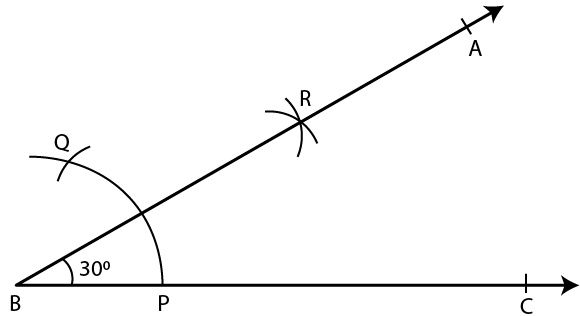(ii) 150

Steps of Construction:

1. Construct a line segment BC.

2. Taking B as centre and a suitable radius construct an arc which meets BC at the point P.

3. Taking P as centre and same radius cut off the arc at the point Q.

4. Consider P and Q as centres, construct two arcs which intersect each other at the point D and join BD.

5. Taking P and R as centre construct two more arcs which intersect each other at the point S.

6. Now join BS and produce it to point A.

∠ABC = 150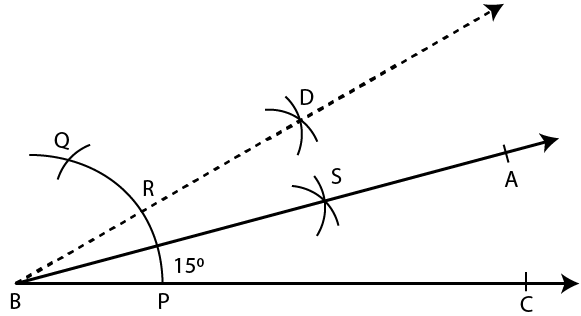(iii) 75°

Steps of Construction:

1. Construct a line segment BC.

2. Taking B as centre and suitable radius construct an arc and cut off PQ then QR of the same radius.

3. Taking Q and R as centre, construct two arcs which intersect each other at the point S.

4. Now join SB.

5. Taking Q and D as centre construct two arcs which intersect each other at the point T.

6. Now join BT and produce it to point A.

∠ABC = 75°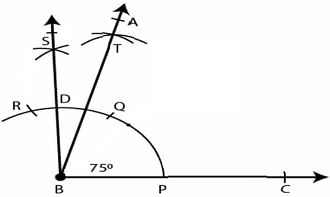(iv) 180°

Steps of Construction:

1. Construct a line segment BC.

2. Taking B as centre and some suitable radius construct an arc which meets BC at the point P.

3. Taking P as centre and with same radius cut off the arcs PQ, QR and RS.

4. Now join BS and produce it to point A.

∠ABC = 180°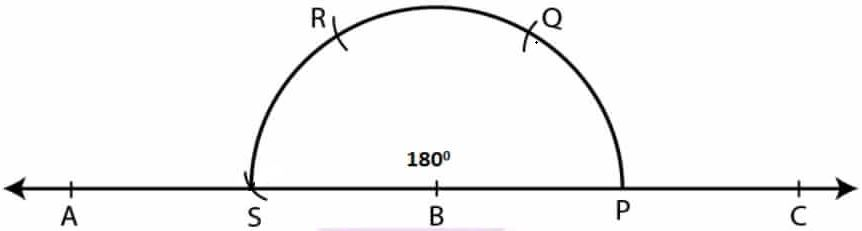(v) 165°

Steps of Construction:

1. Construct a line segment BC.

2. Taking B as centre and some suitable radius construct an arc which meets BC at the point P.

3. Taking P as centre and same radius cut off arcs at PQ, QR and then RS.

4. Now join SB and produce it to point E.

5. Taking R and S as centres construct two arcs which intersect each other at the point M.

6. Taking T and S as centres construct two arcs which intersect each other at the point L.

7. Now join BL and produce it to point A.

∠ABC = 165°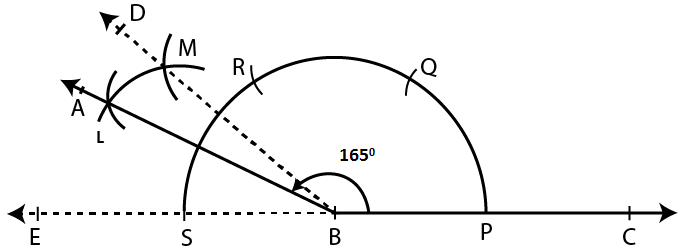2. Draw ∠ABC = 120°. Bisect the angle using ruler and compasses only. Measure each angle so obtained and check whether the angles obtained on bisecting ∠ABC are equal or not.

Solution:

Steps of Construction:

1. Construct a line segment BC.

2. Taking B as centre and some suitable radius construct an arc which meets BC at the point P.

3. Taking P as centre and with same radius cut off the arcs PQ and QR.

4. Now join BR and produce it to point A

∠ABC = 120°

5. Taking P and R as centres construct two arcs which intersect each other at the point S.

6. Now join BS and produce it to point D.

Here BD is the bisector of ∠ABC

By measuring each angle we get to know that is it 600

Yes, both the angles are of equal measure.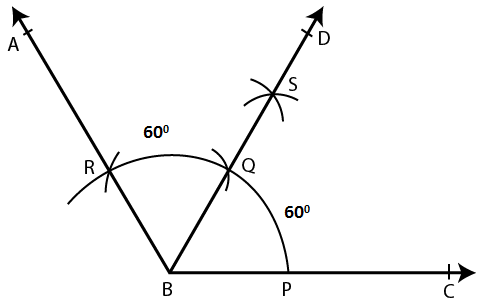3. Draw a line segment PQ = 6 cm. Mark a point A in PQ so that AP = 2 cm. At point A, construct angle QAR = 60°.

Solution:

Steps of Construction:

1. Construct a line segment PQ = 6cm.

2. Now mark point A on PQ so that AP = 2 cm.

3. Taking A as centre and some suitable radius construct an arc which meets AQ at the point C.

4. Taking C as centre and with same radius cut the arc CB.

5. Now join AB and produce it to point R.

∠QAR = 600.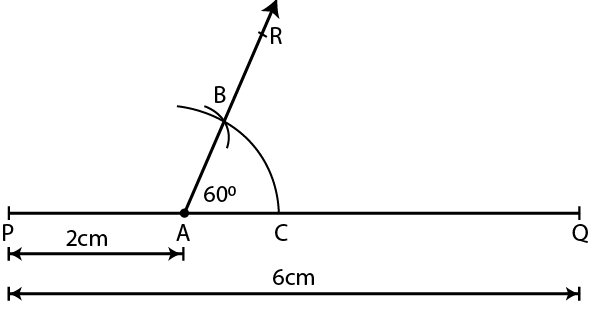4. Draw a line segment AB = 8 cm. Mark a point P in AB so that AP = 5 cm. At P, construct angle APQ = 30°.

Solution:

Steps of Construction:

1. Construct a line segment AB = 8 cm.

2. Now mark the point P on AB such that AP = 5 cm.

3. Taking P as centre and some suitable radius construct an arc which meets AB at L.

4. Taking L as centre and same radius cut the arc LM.

5. Now bisect the arc LM at the point N.

6. Join PN and produce it to point Q.

∠APQ = 300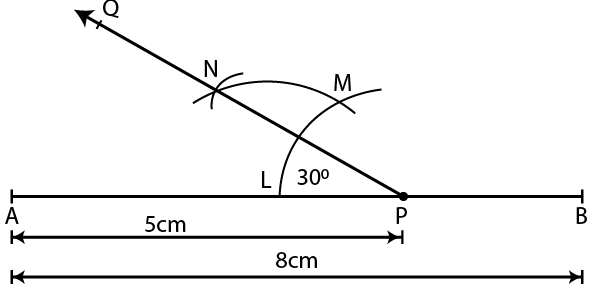5. Construct an angle of 75° and then bisect it.

Solution:

Steps of Construction:

1. Construct a line segment BC.

2. At the point B construct an angle ABC which is equal to 750.

3. Taking P and T as centres construct arcs which intersect each other at the point L.

4. Now join BL and produce it to point D.

Here BD bisects ∠ABC.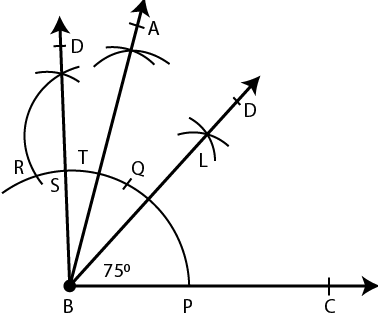6. Draw a line segment of length 6 .4 cm. Draw its perpendicular bisector.

Solution:

Steps of Construction:

1. Construct a line segment AB = 6.4 cm.

2. Taking A and B as centres and with some suitable radius construct arcs which intersect each other at the points S and R.

3. Now join SR which intersects AB at the point Q.

Here PQR is the perpendicular bisector of the line segment AB.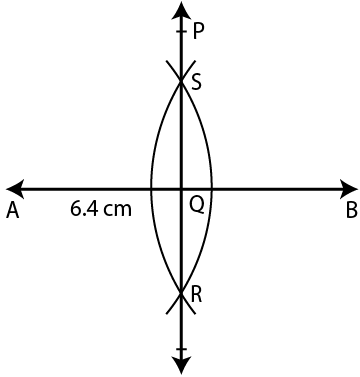7. Draw a line segment AB = 5.8 cm. Mark a point P in AB such that PB = 3.6 cm. At P, draw perpendicular to AB.

Solution:

Steps of Construction:

1. Construct a line segment AB = 5.8 cm.

2. Now mark a point P on the line segment AB such that PB = 3.6 cm.

3. Taking P as centre and some suitable radius construct an arc which meets AB at the point L.

4. Taking L as centre and same radius cut off the arcs LM and  MN.

5. Now bisect the arc MN at the point S.

6. Join PS and produce it to point Q.

Here PQ is perpendicular to the line segment AB at point P.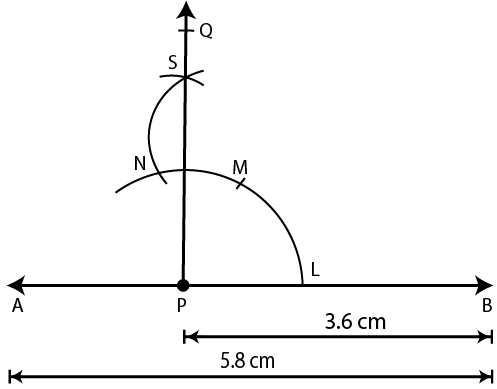8. In each case, given below, draw a line through point P and parallel to AB: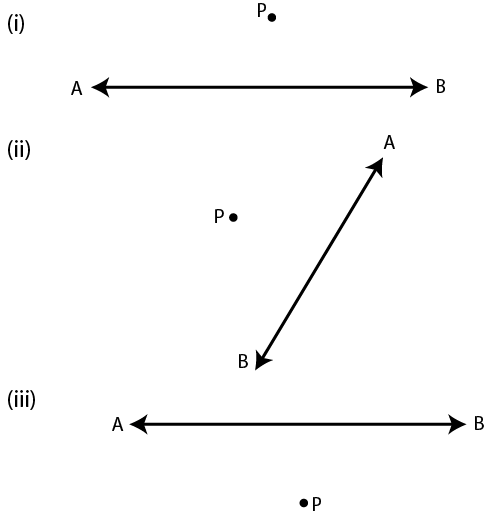Solution:

Steps of Construction:

1. From the point P construct a line segment meeting AB

2. Taking Q as centre and some suitable radius construct an arc CD.

3. Taking P as centre and same radius construct another arc which meets PQ at the point E.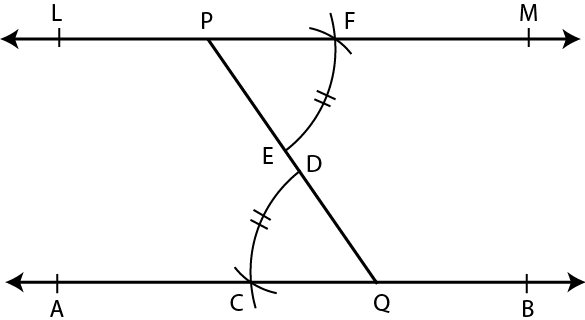4. Taking E as centre and radius equal to CD cut this arc at the point F.5. Now join PF and produce it to both sides to L and M.

Here the line LM is parallel to the given line AB.